# R S Aggarwal Solutions for Class 10 Maths Chapter 8 Circles

R S Aggarwal Solutions for Class 10 is a beneficial solution guide prepared by subject experts at BYJU’S to help students clear their doubts. Chapter 8 of class 10 is loaded with various exercises that are board and competitive exam-oriented. The set of questions and answers will guide students in getting acquainted with the concepts. Find more concepts on R S Aggarwal Solutions for Maths designed by experts to help students learn the most efficient way possible. Students can avail the R S Aggarwal Solutions and download the pfd for free.

## Download PDF of R S Aggarwal Solutions for Class 10 Maths Chapter 8 Circles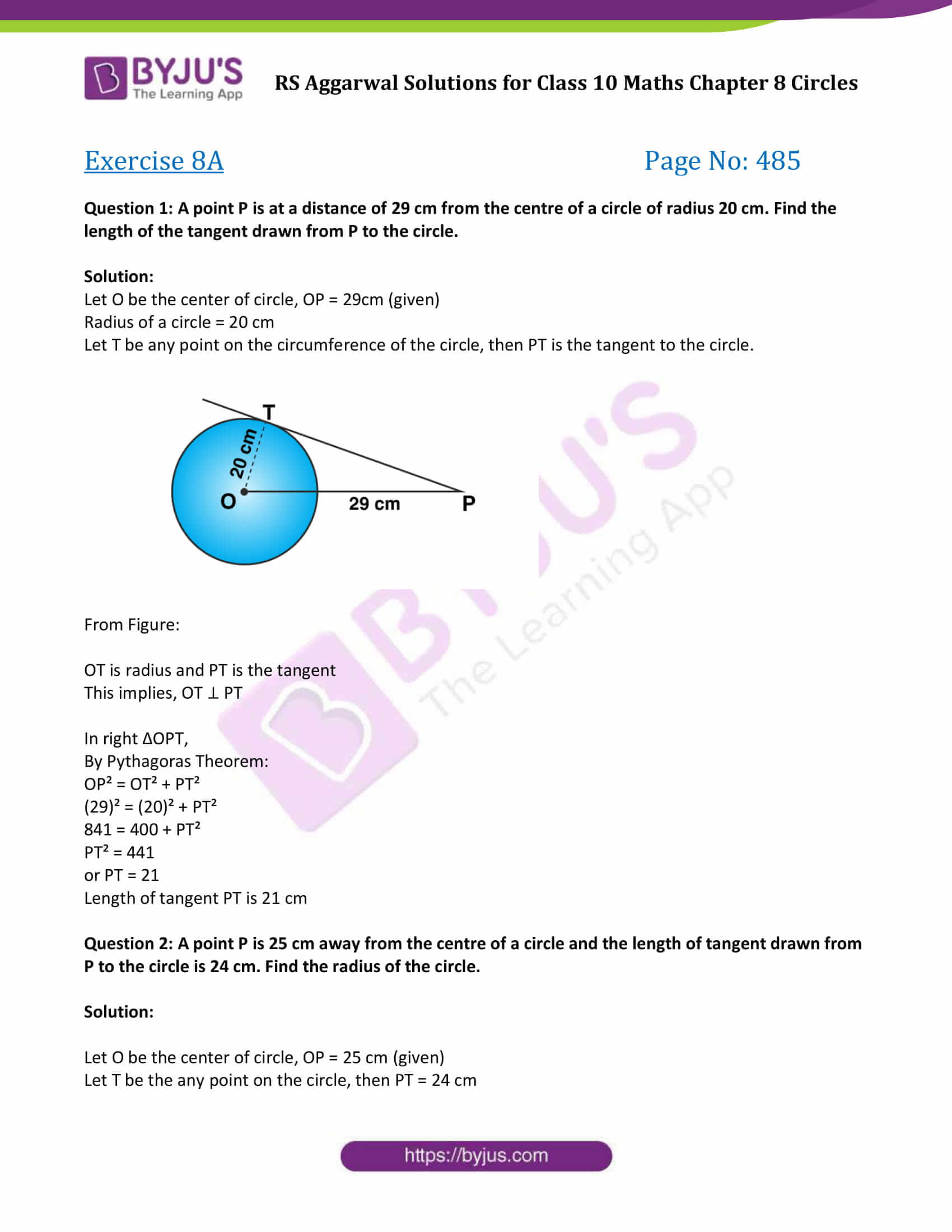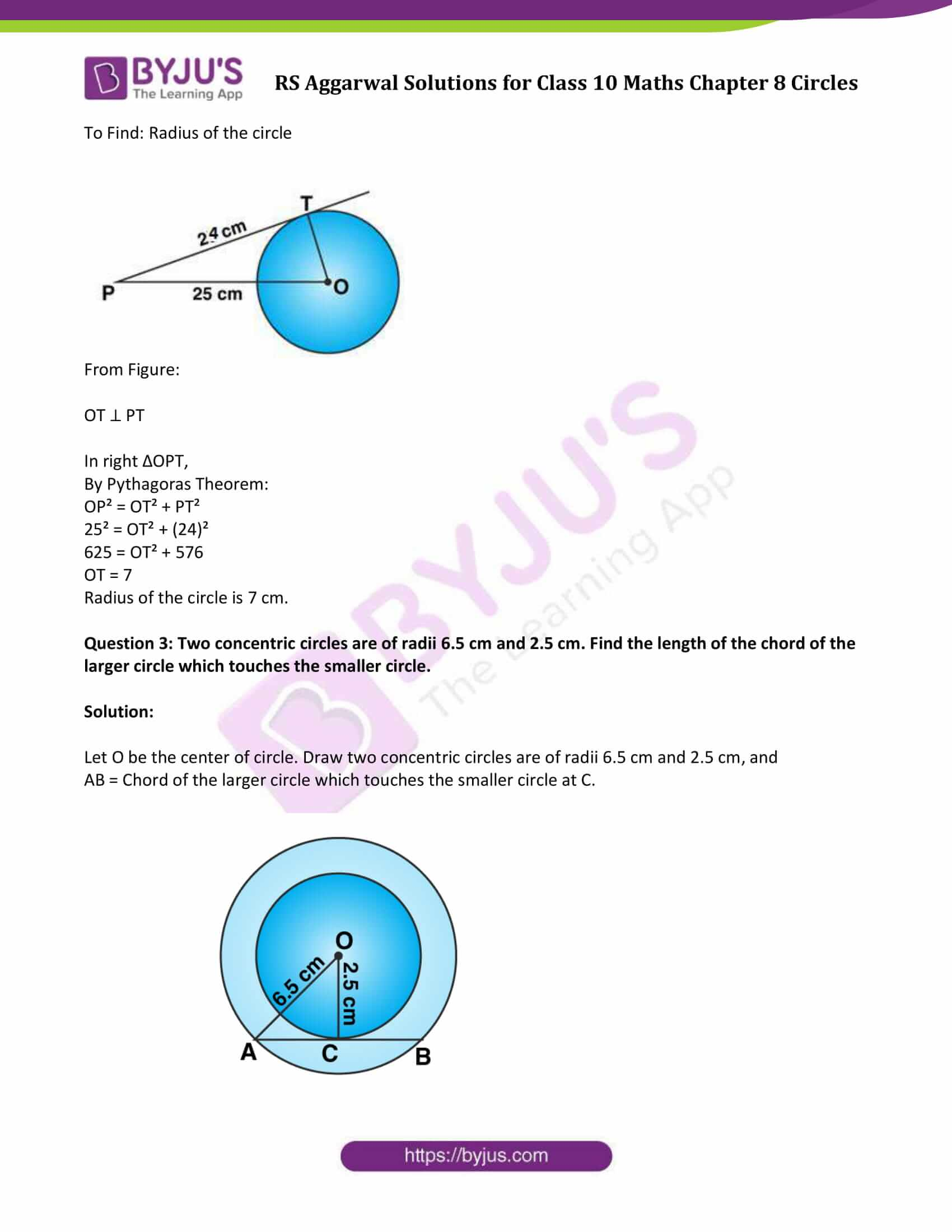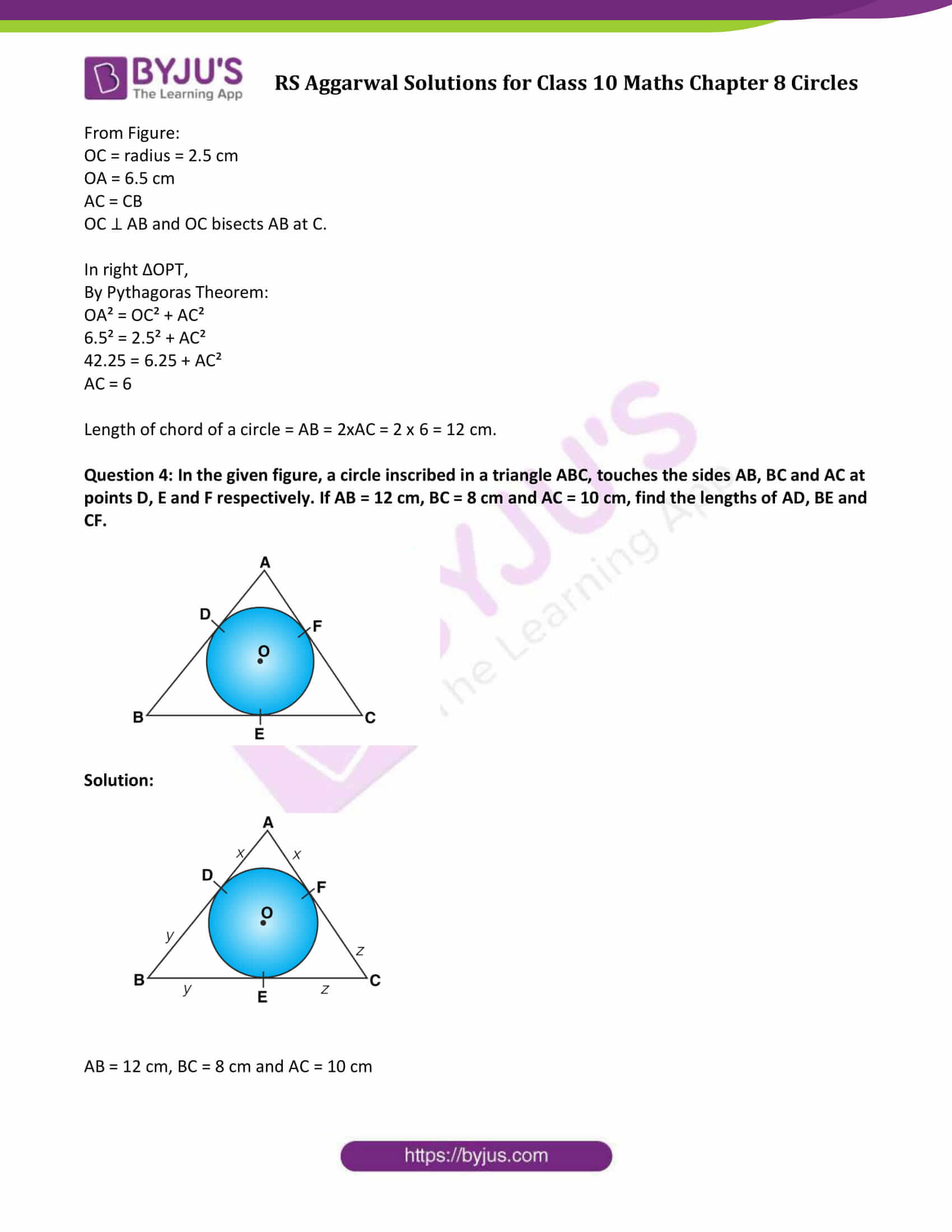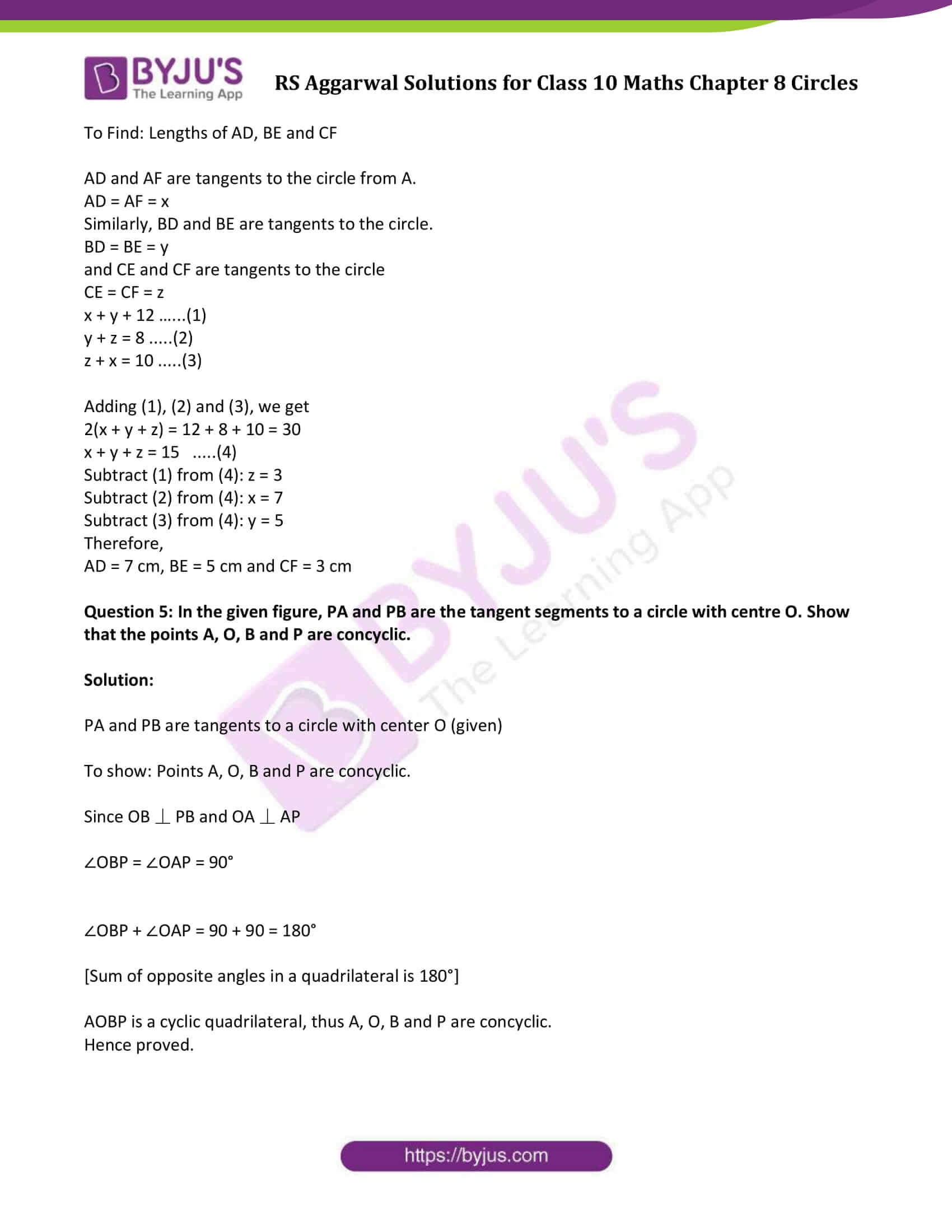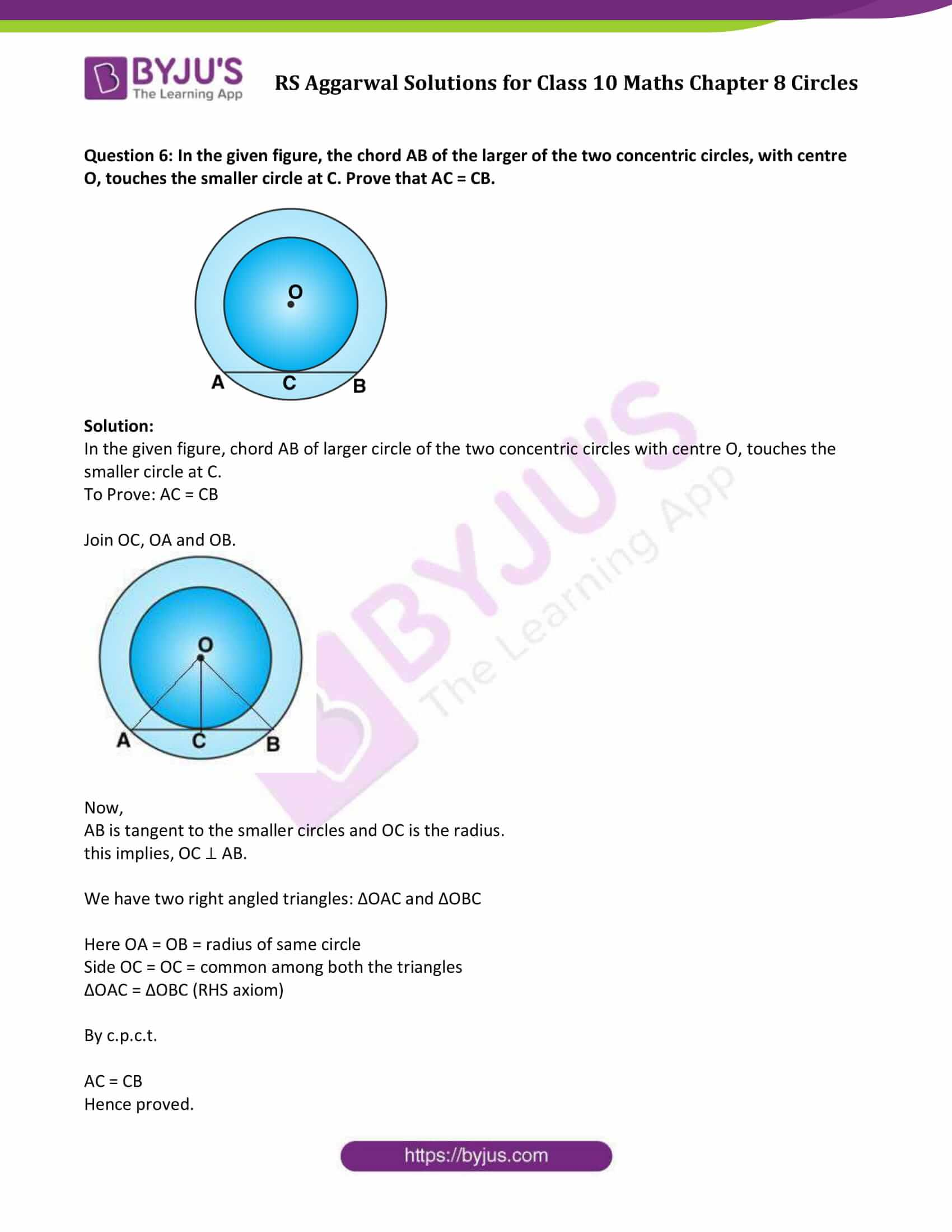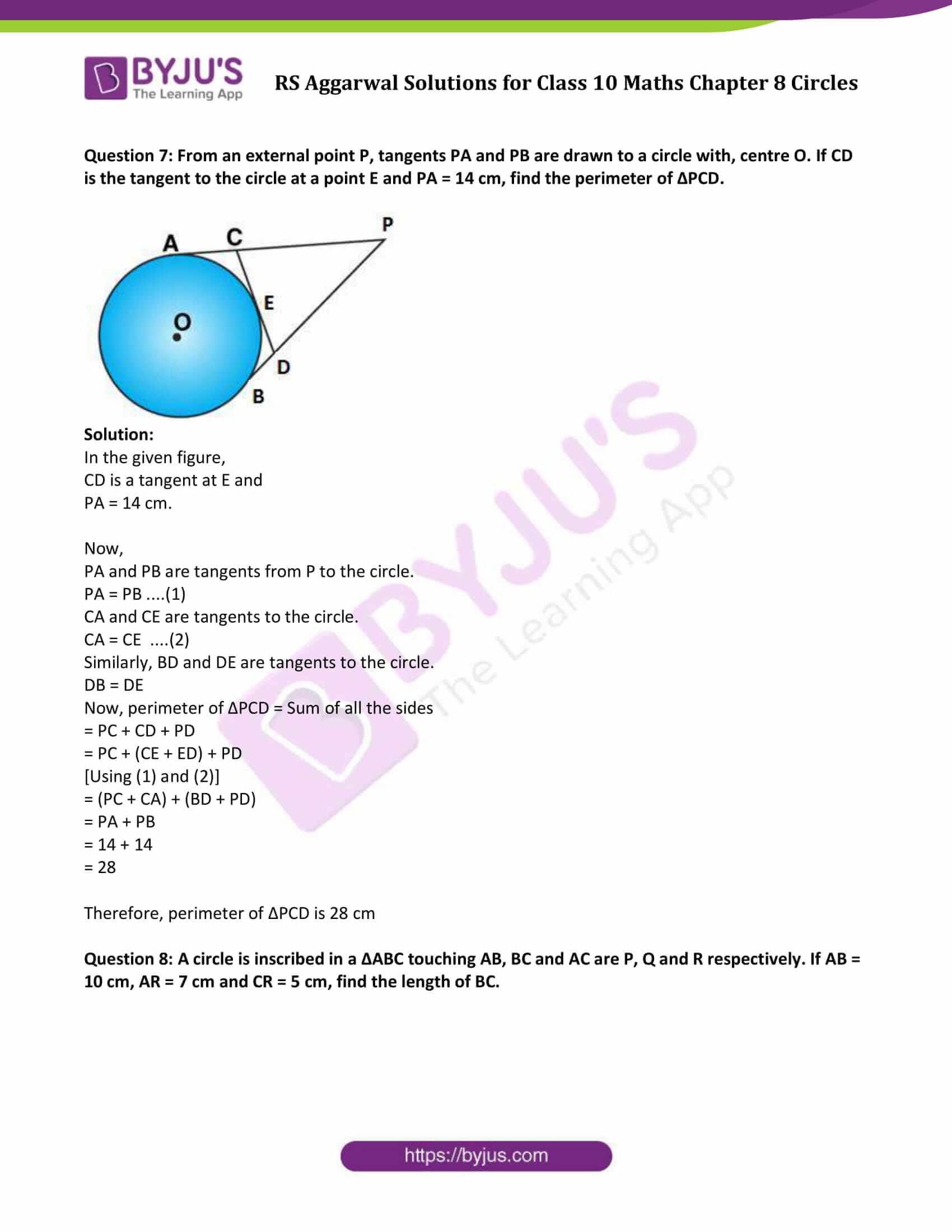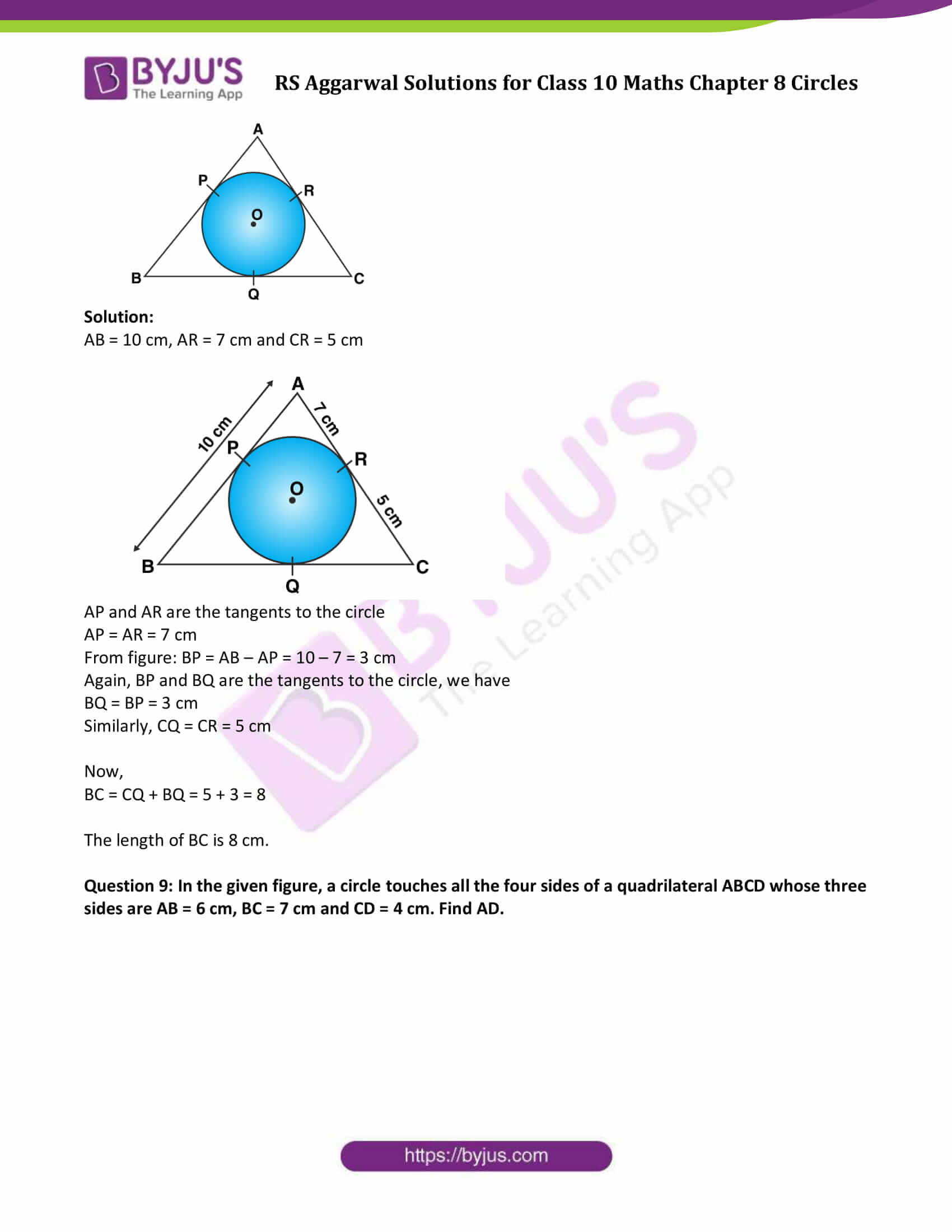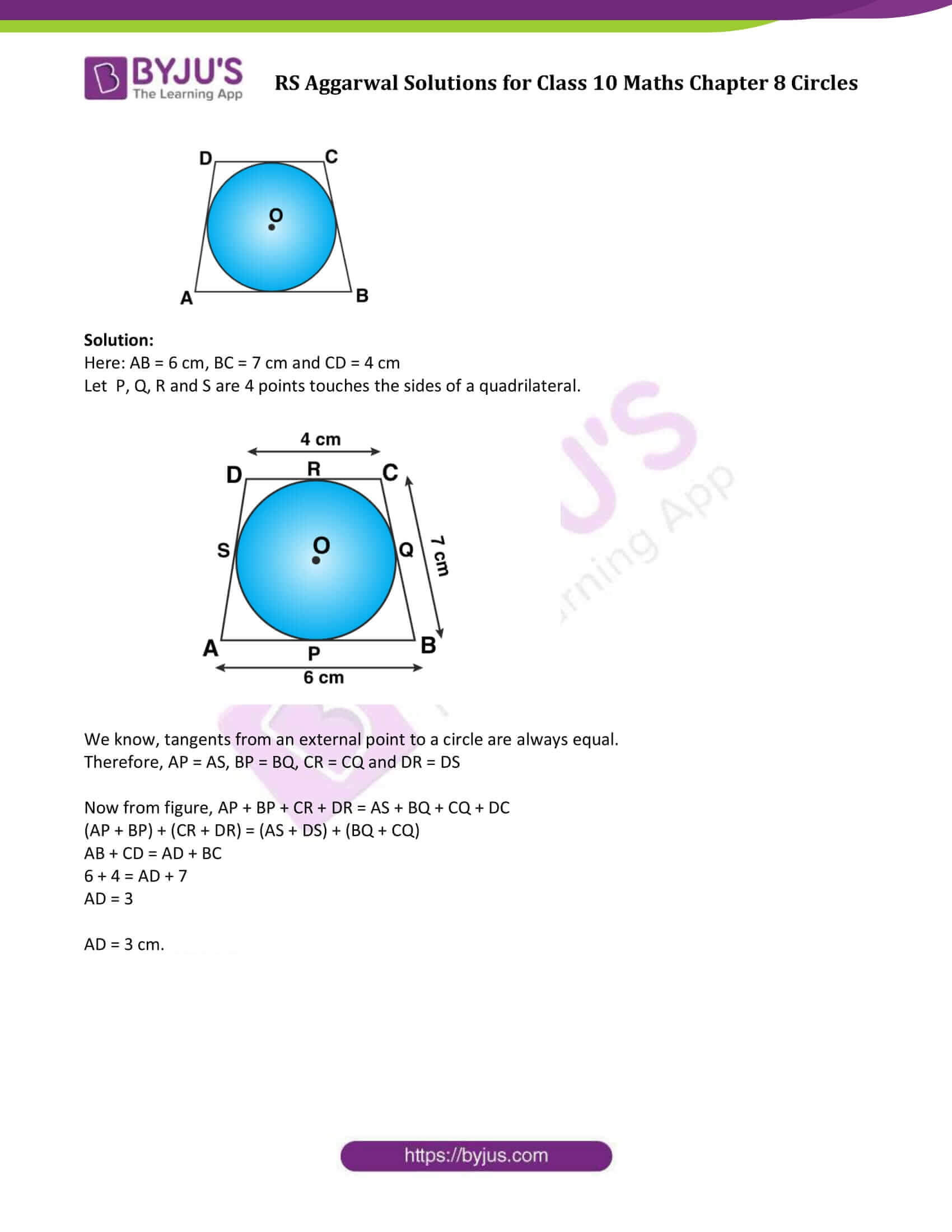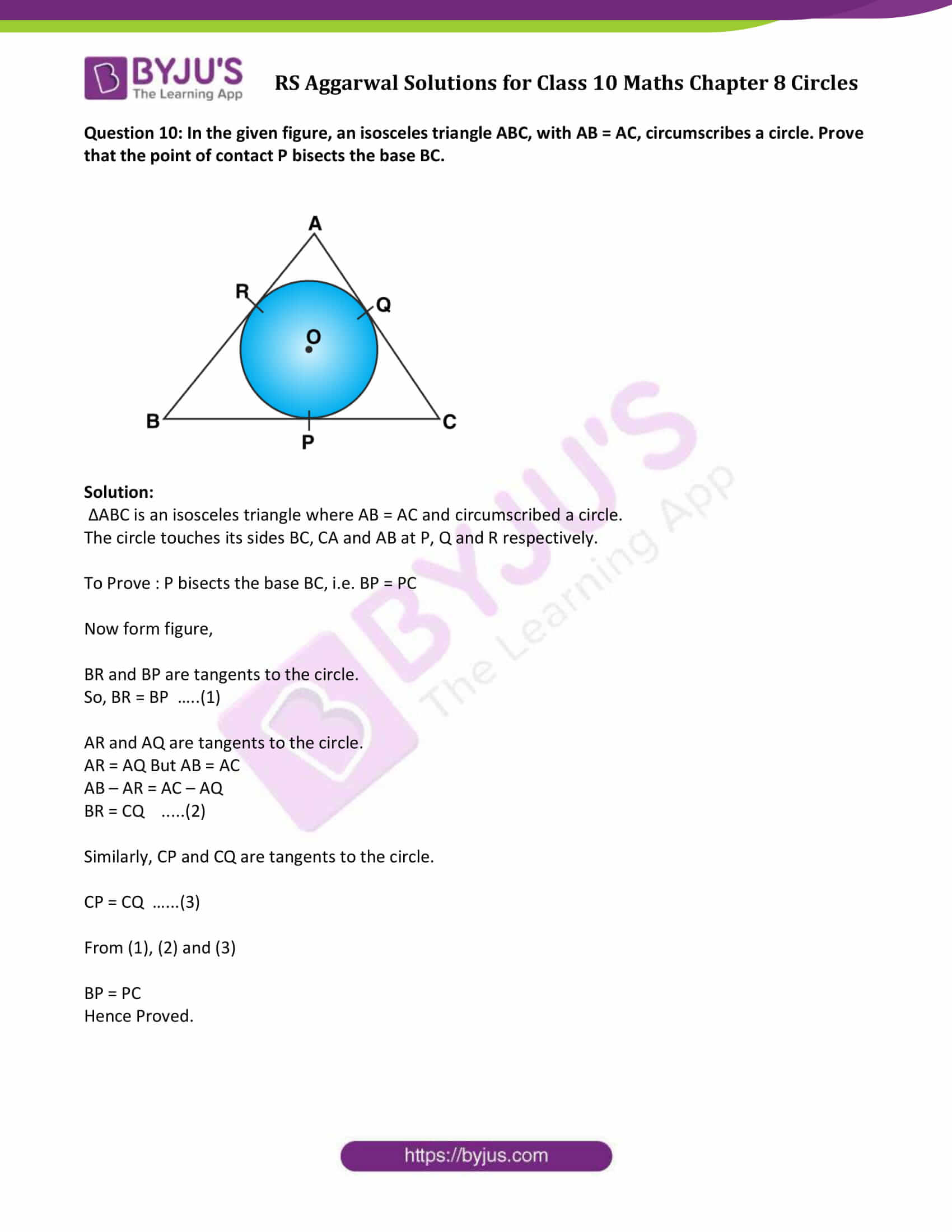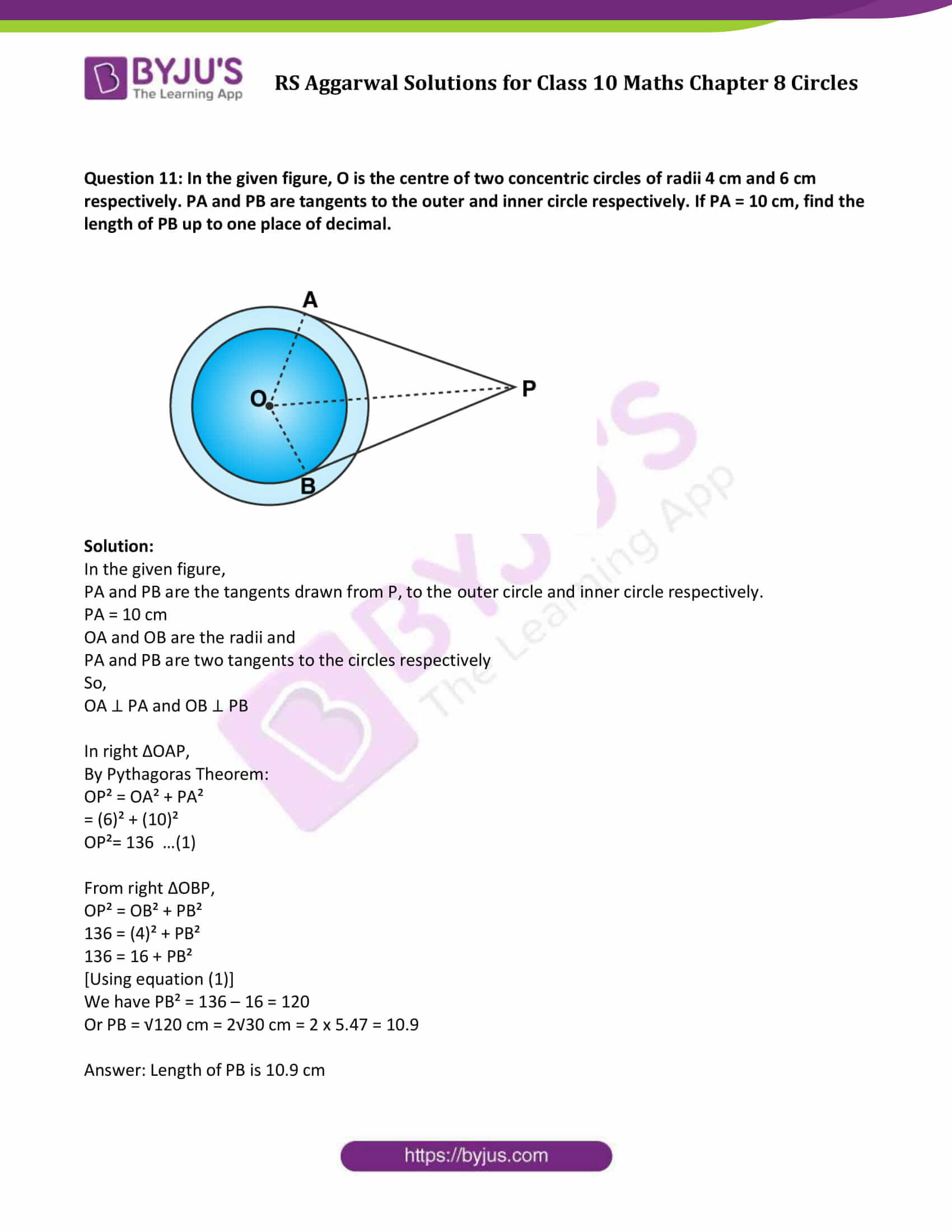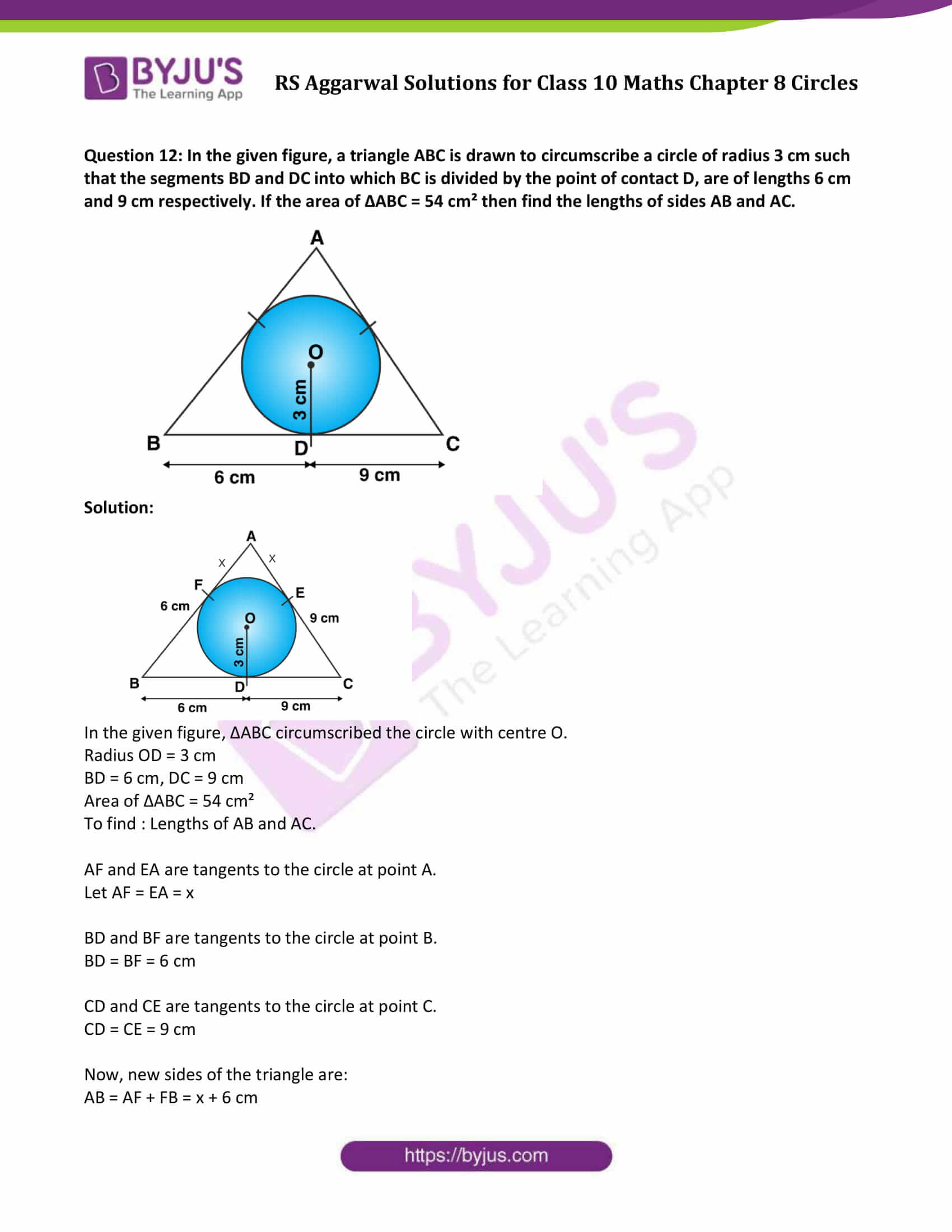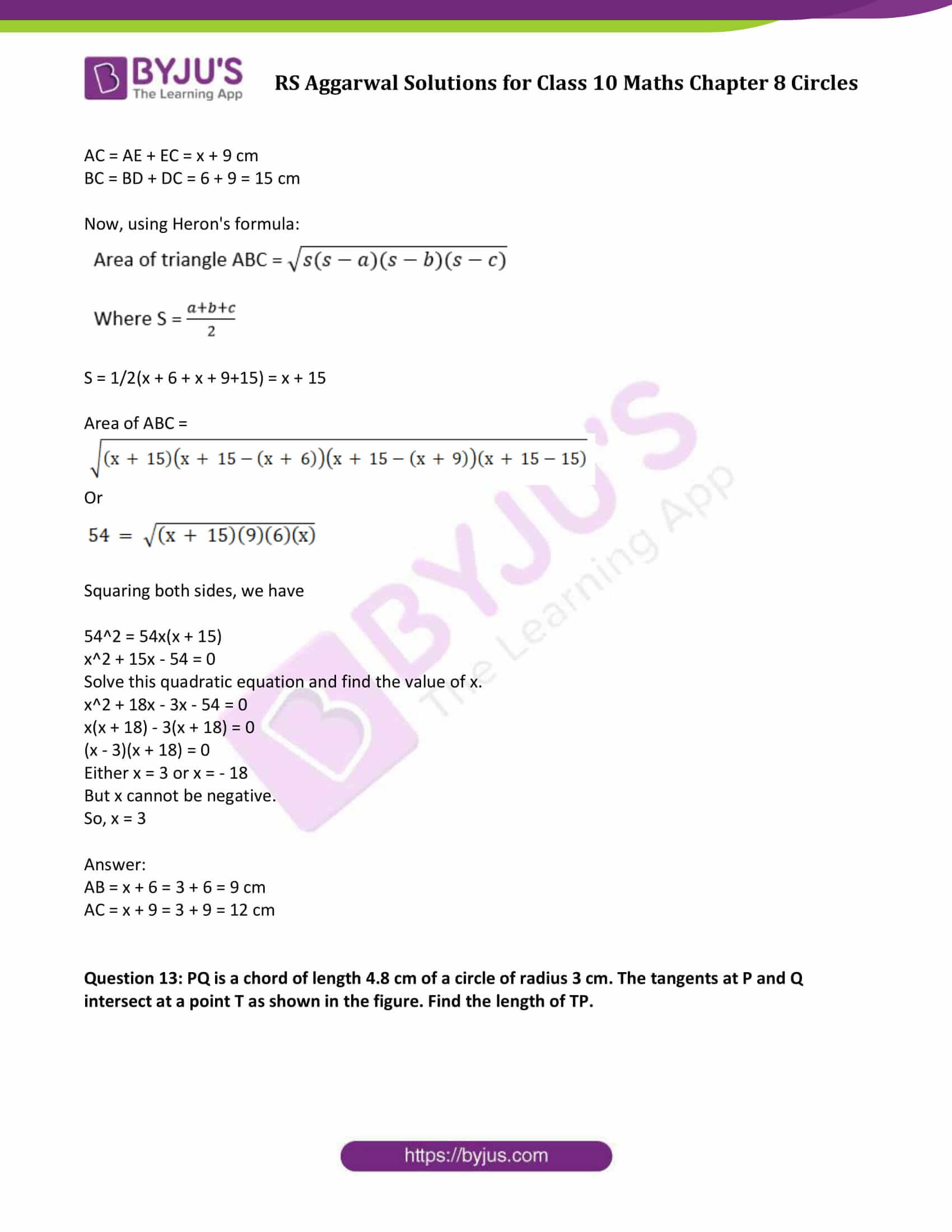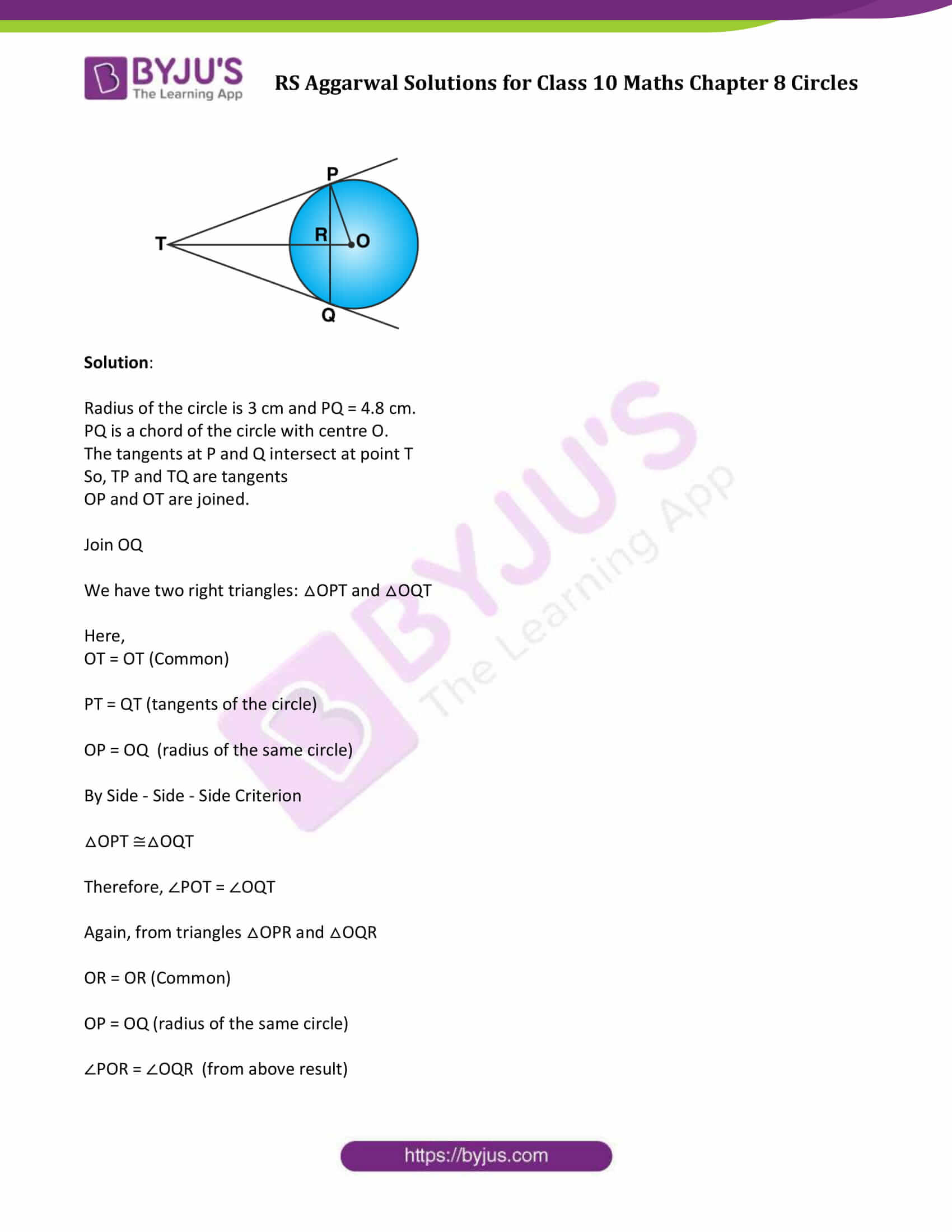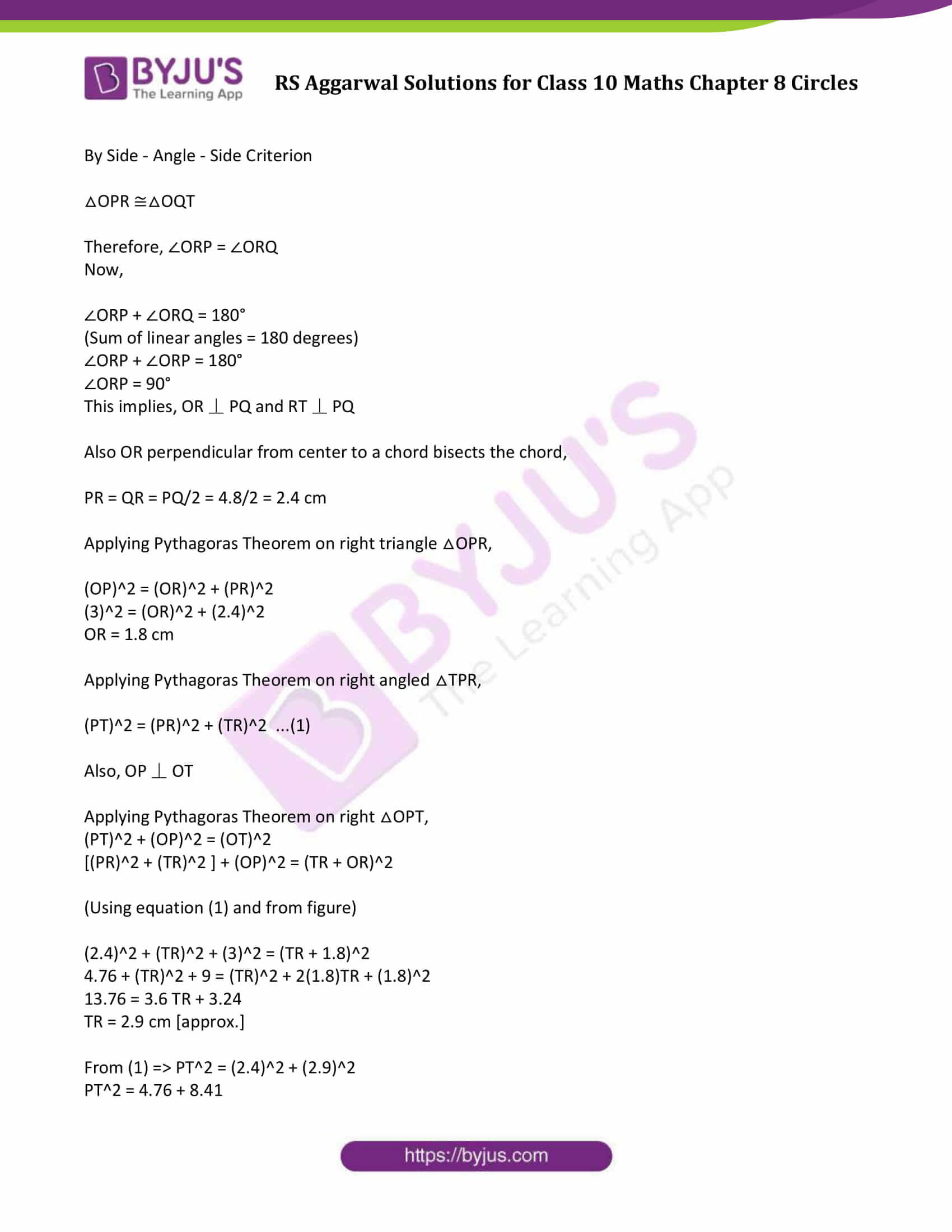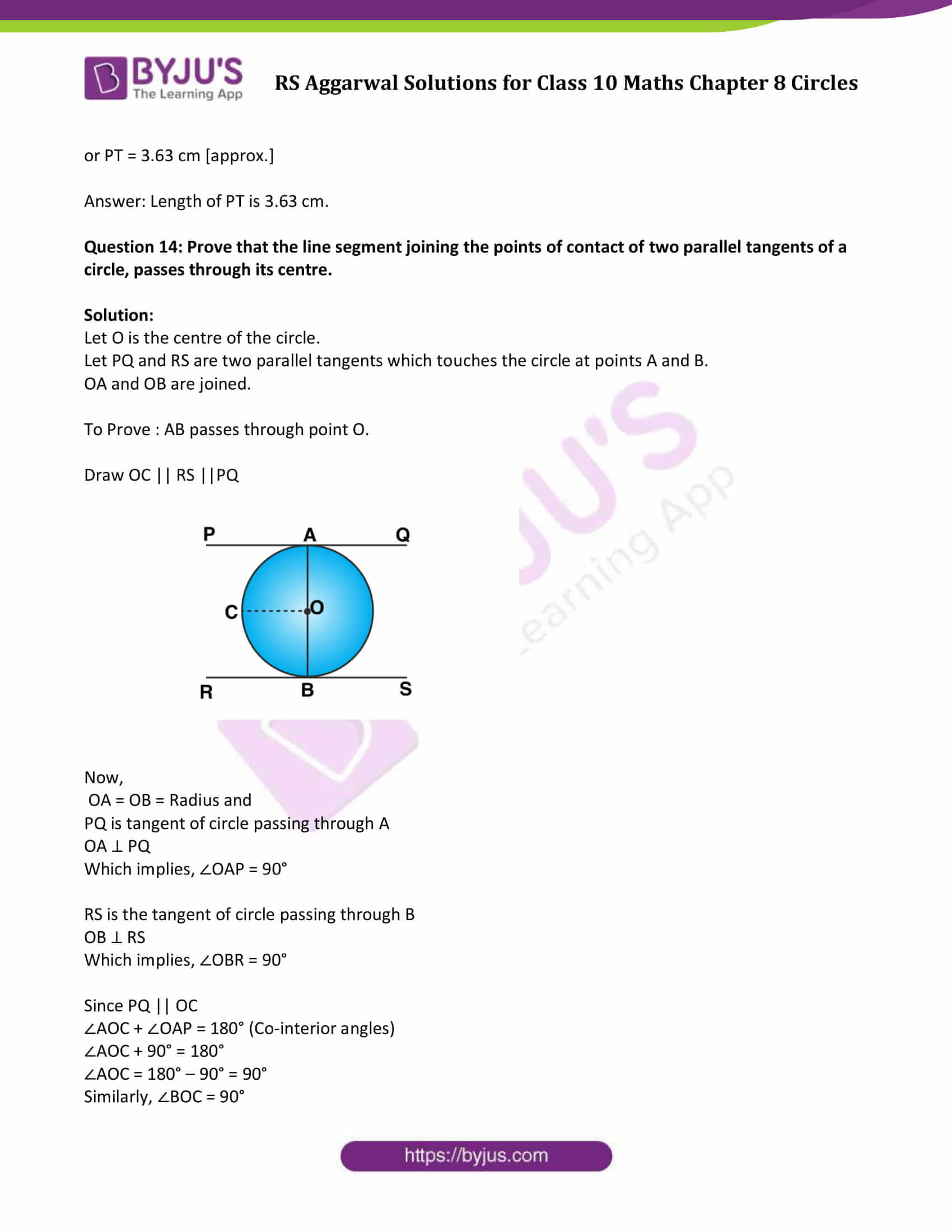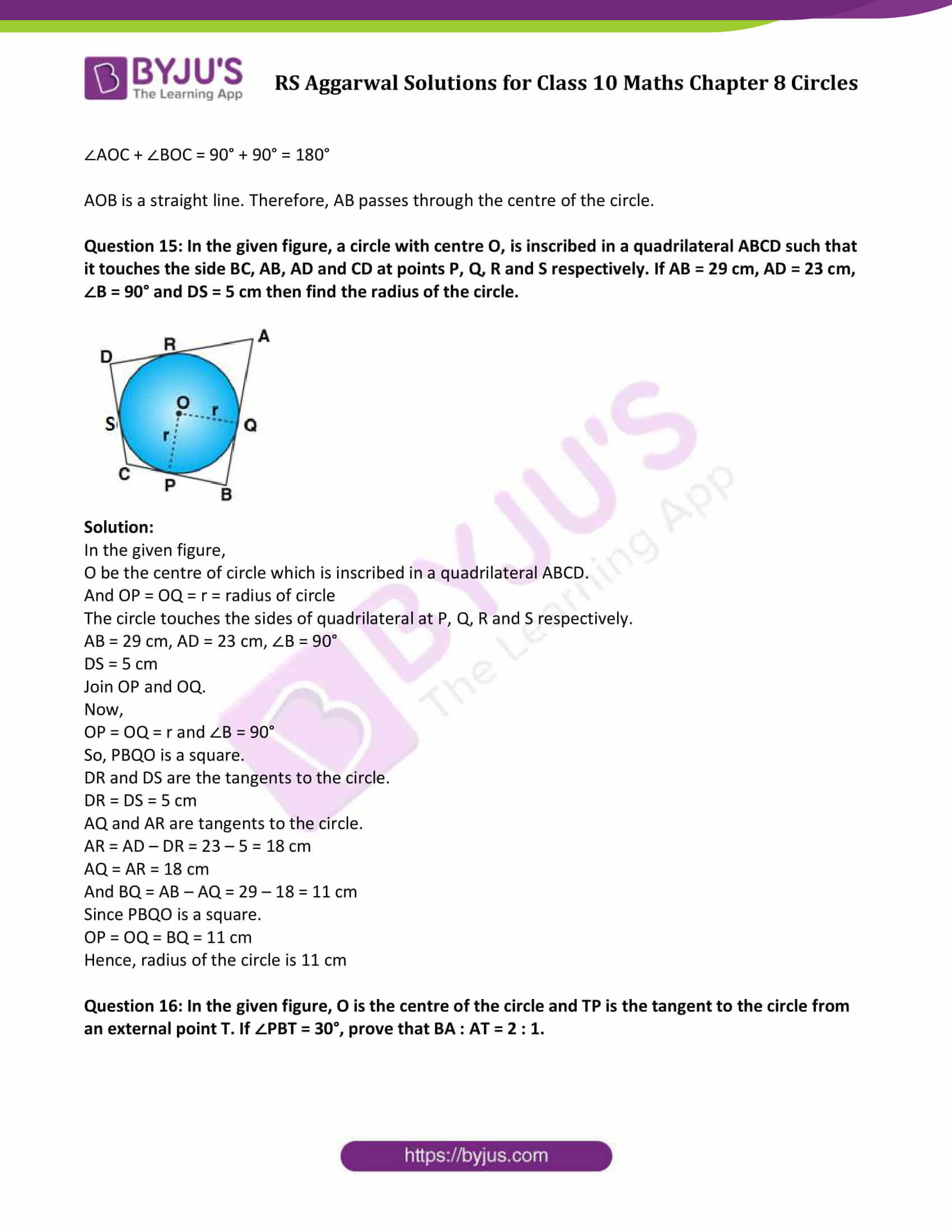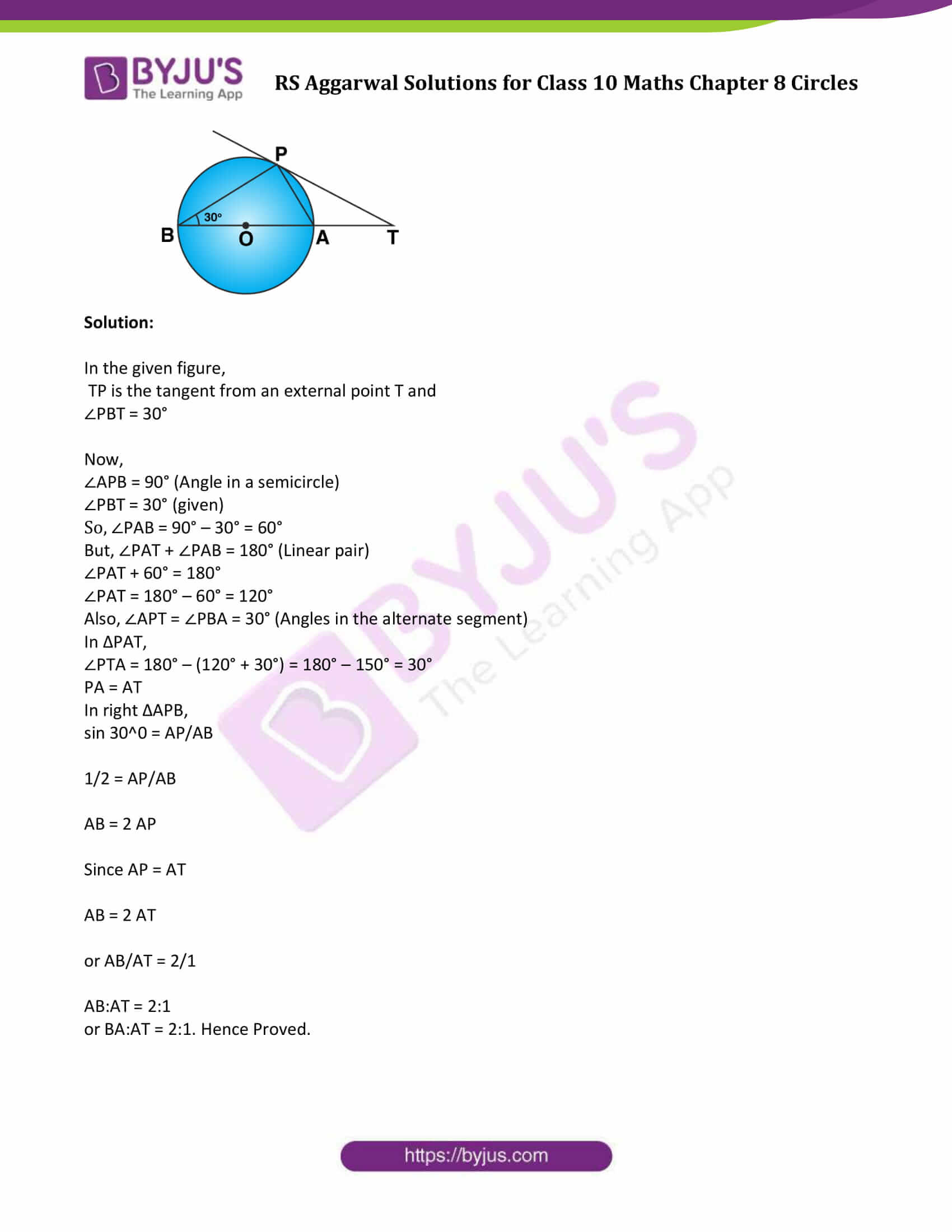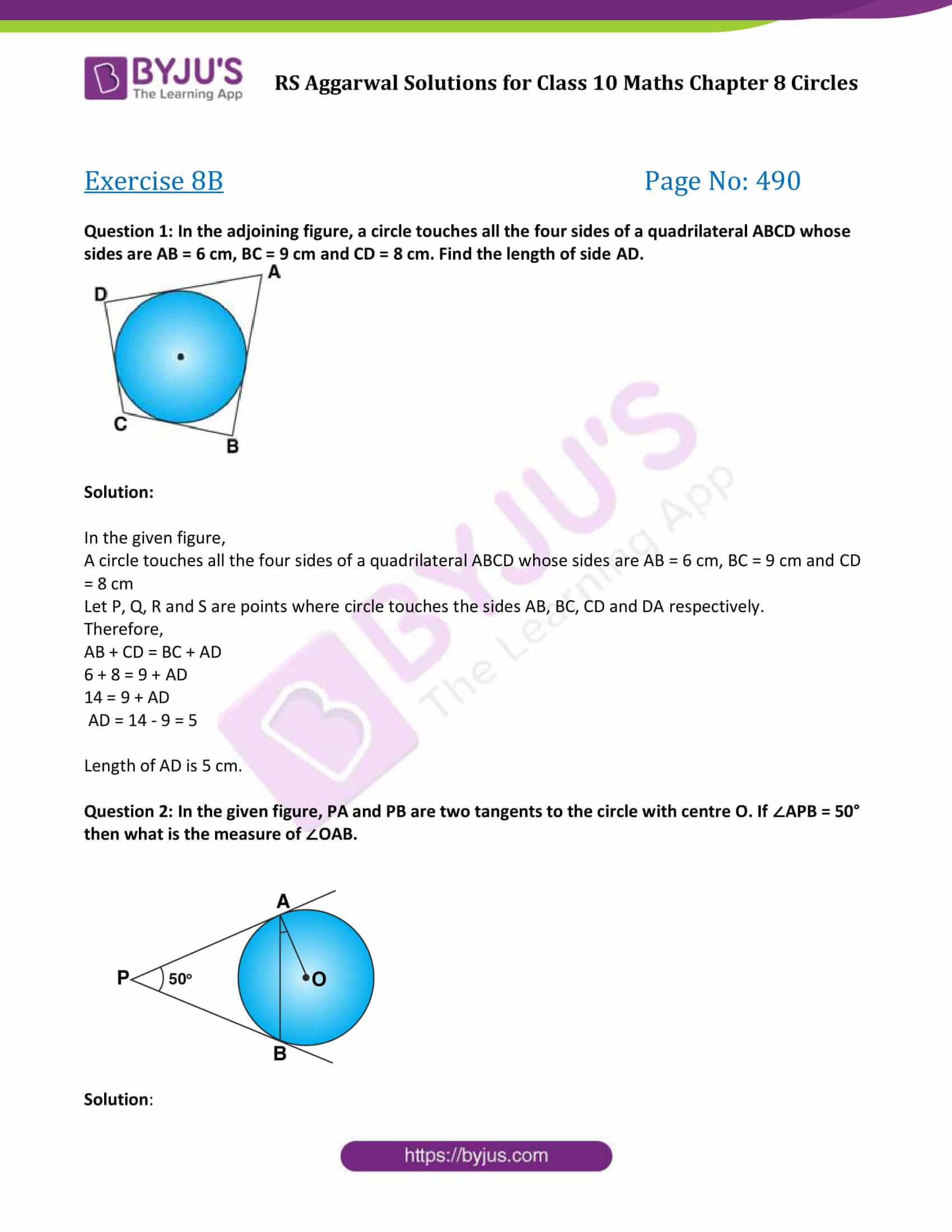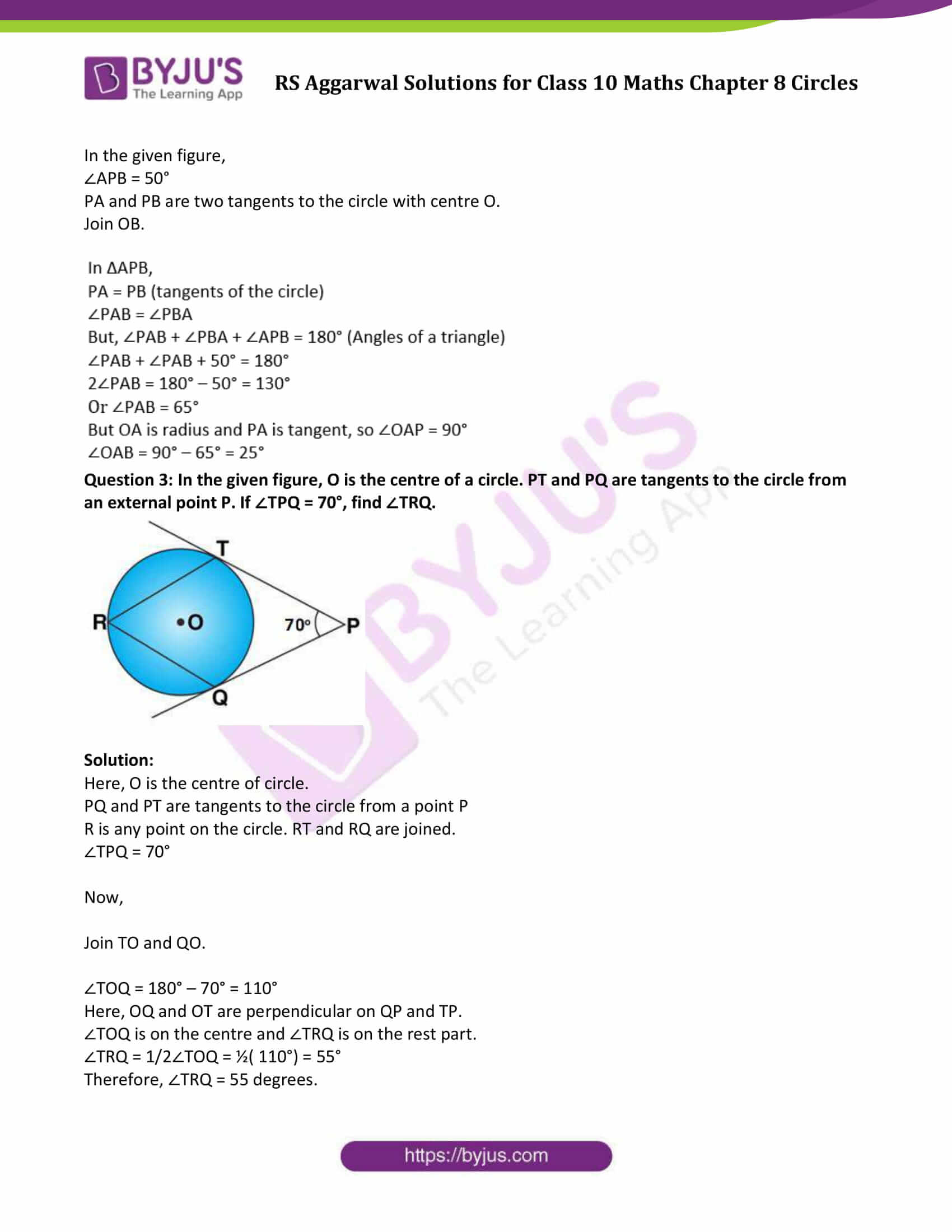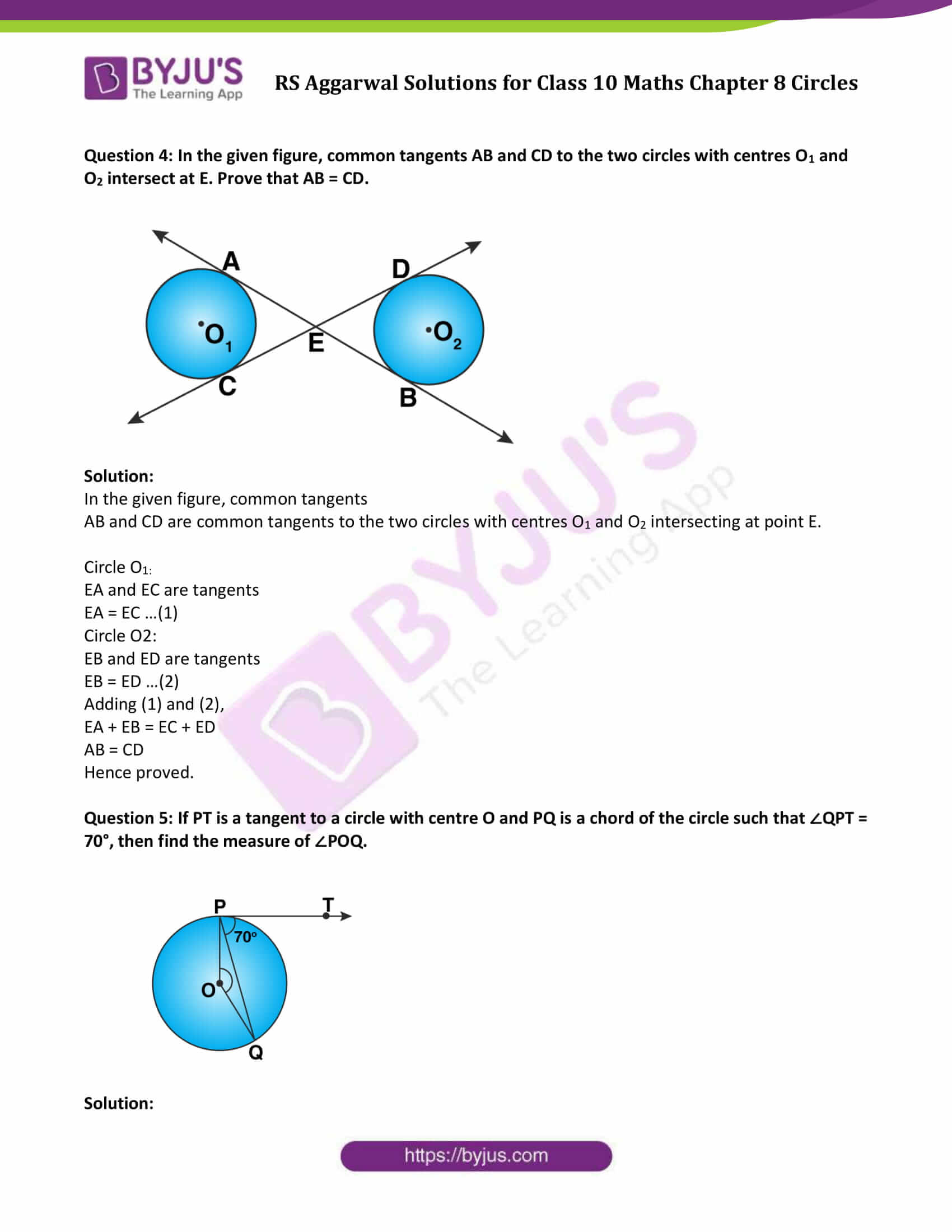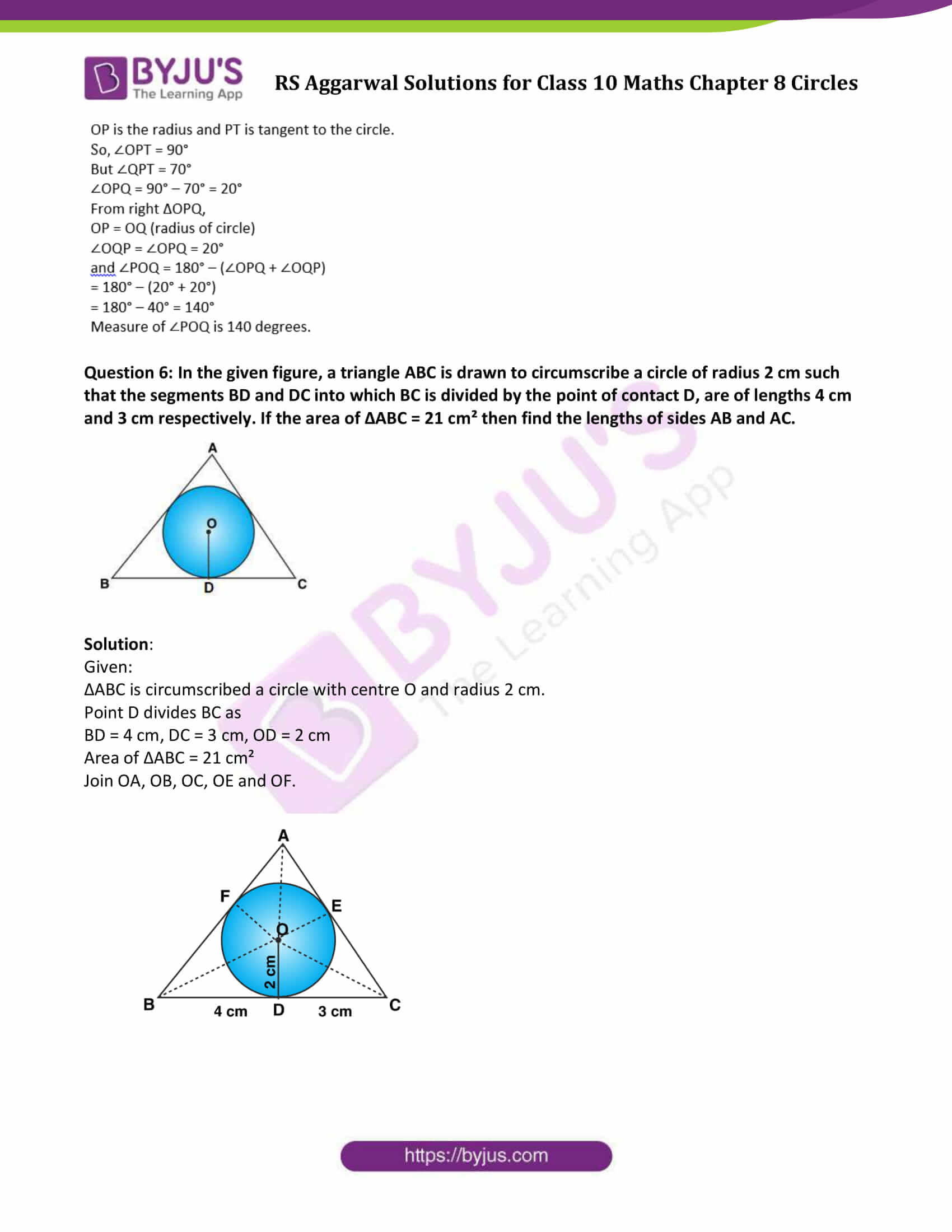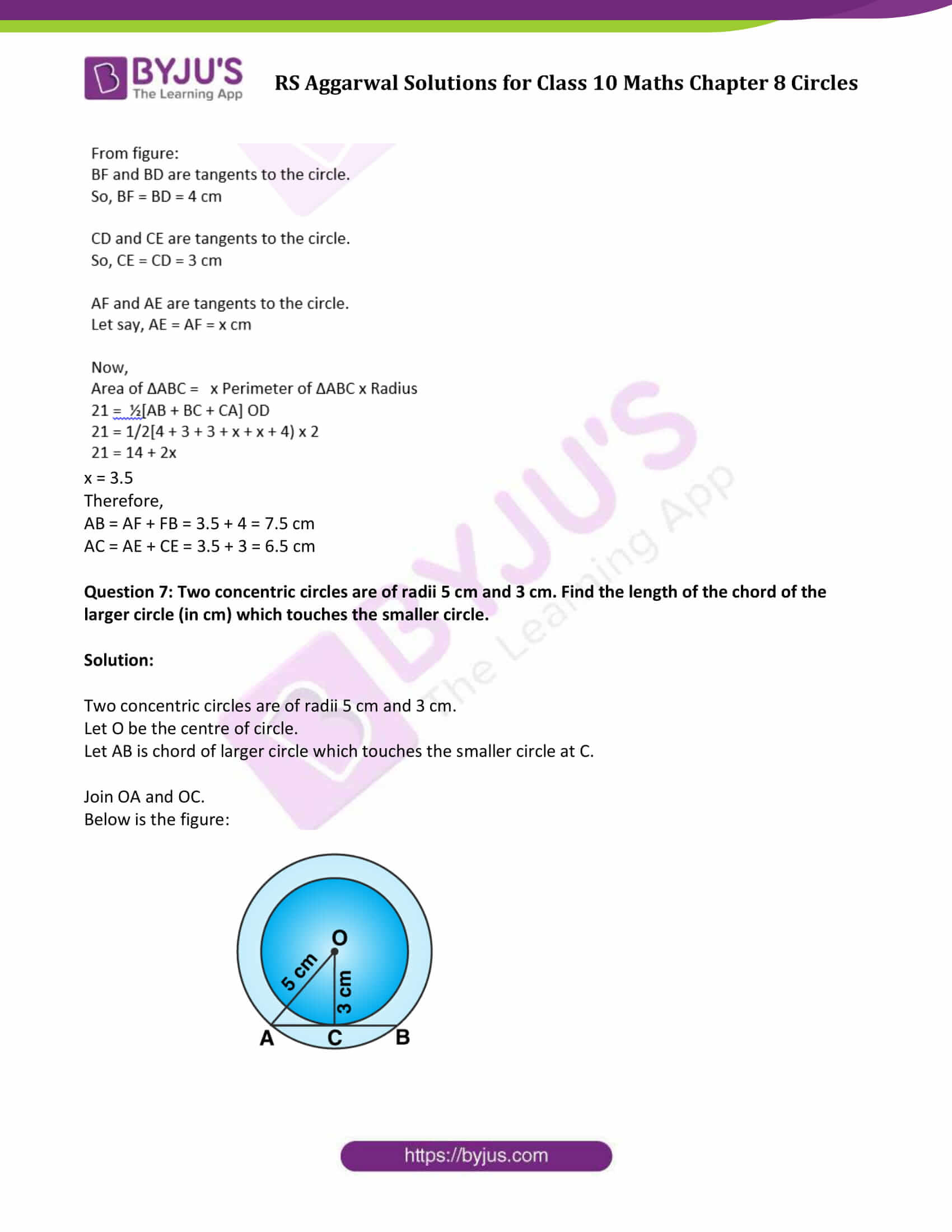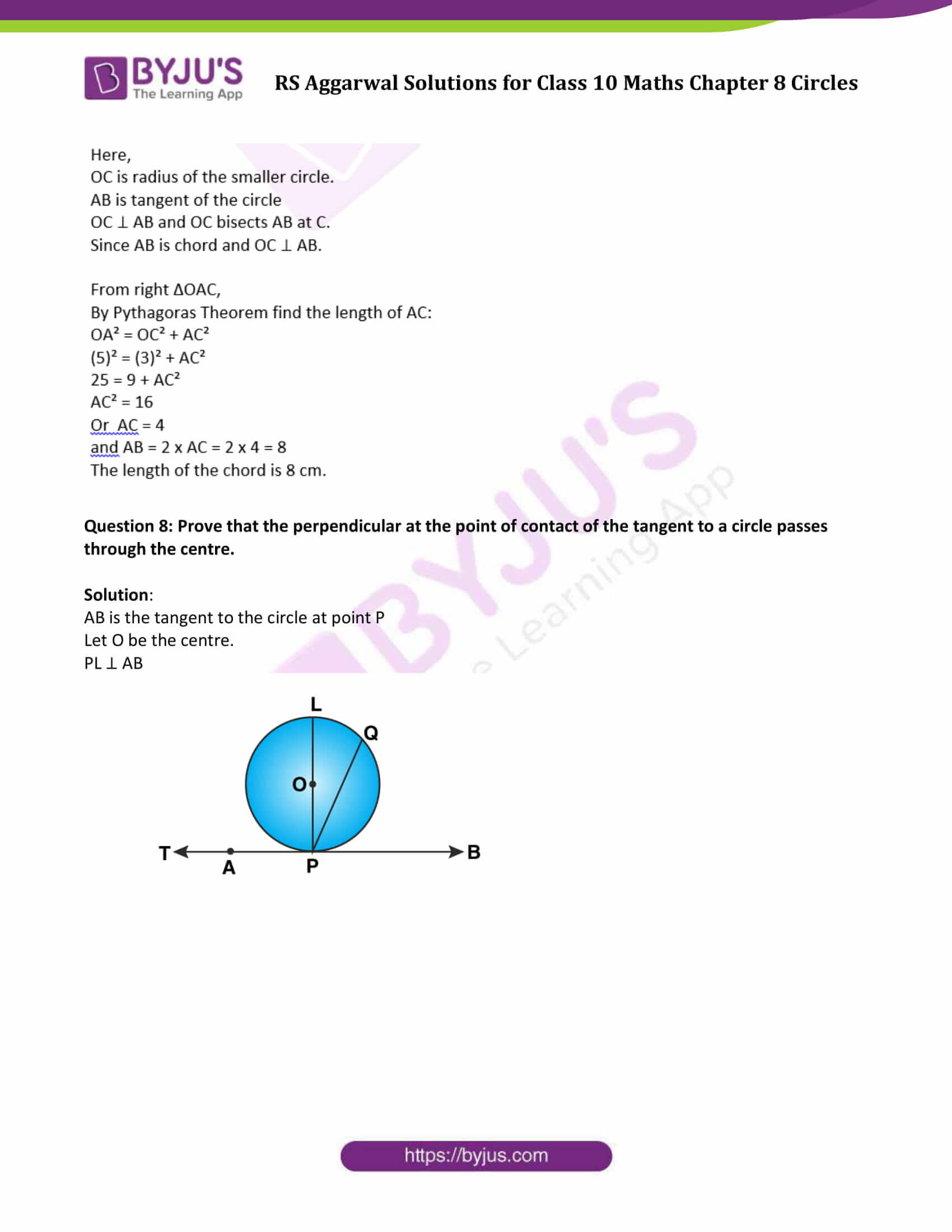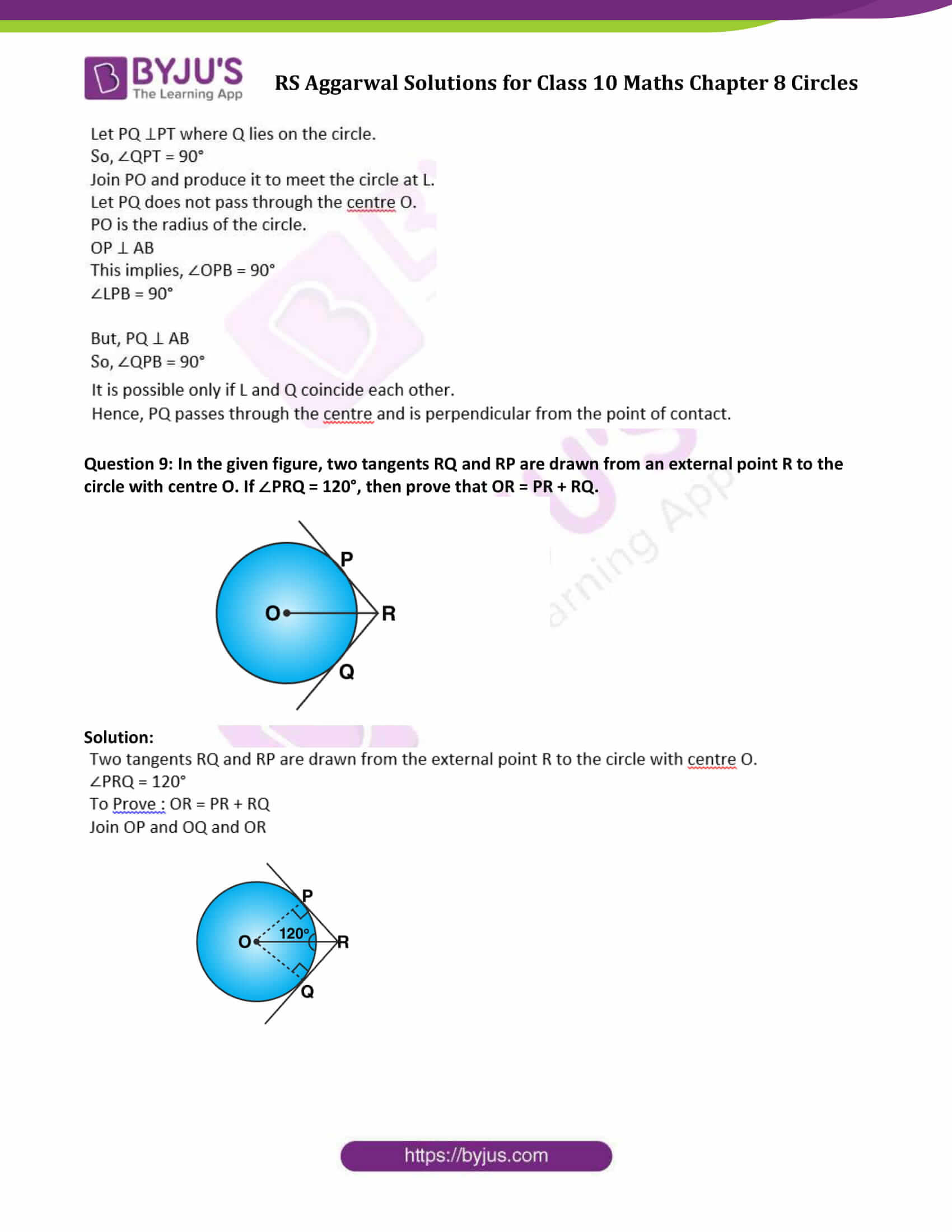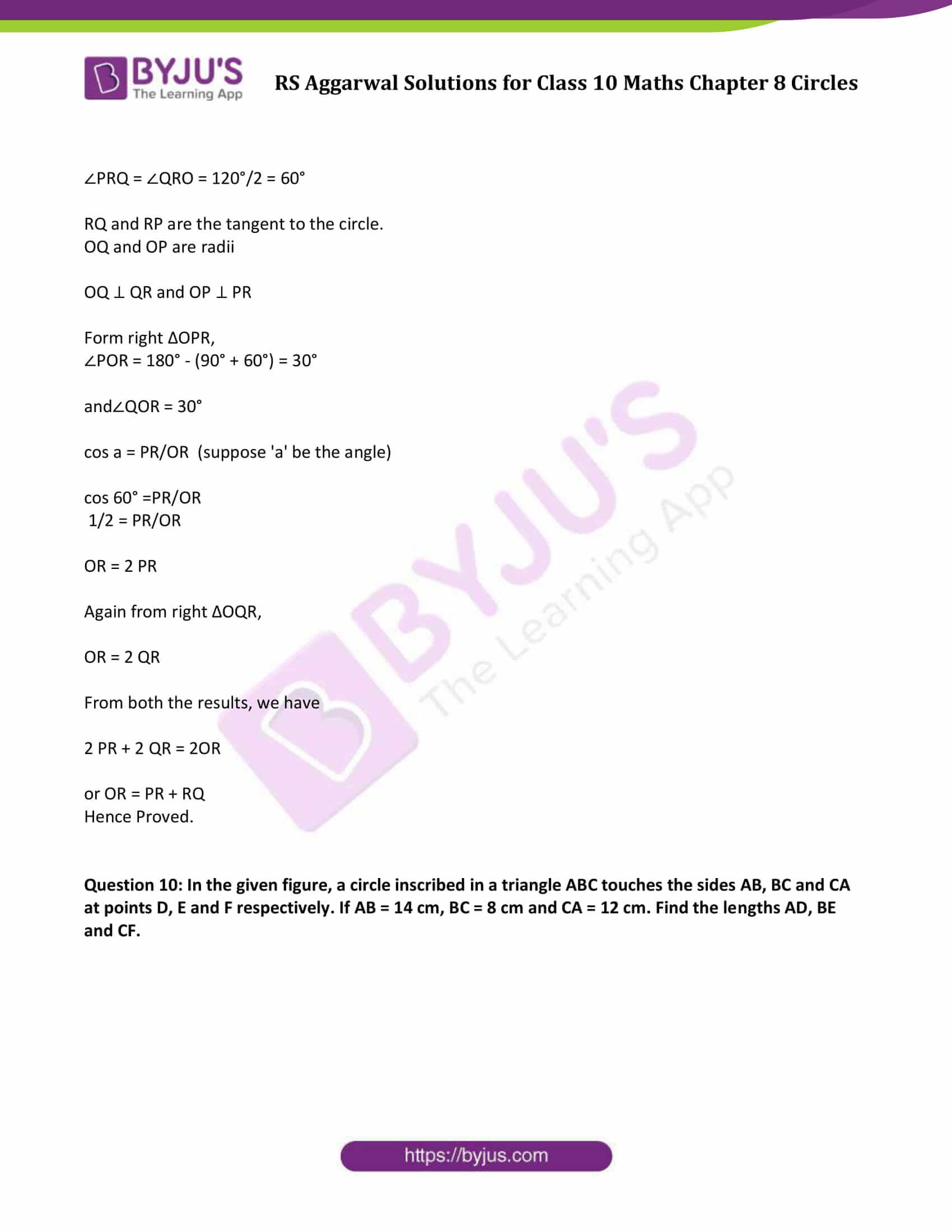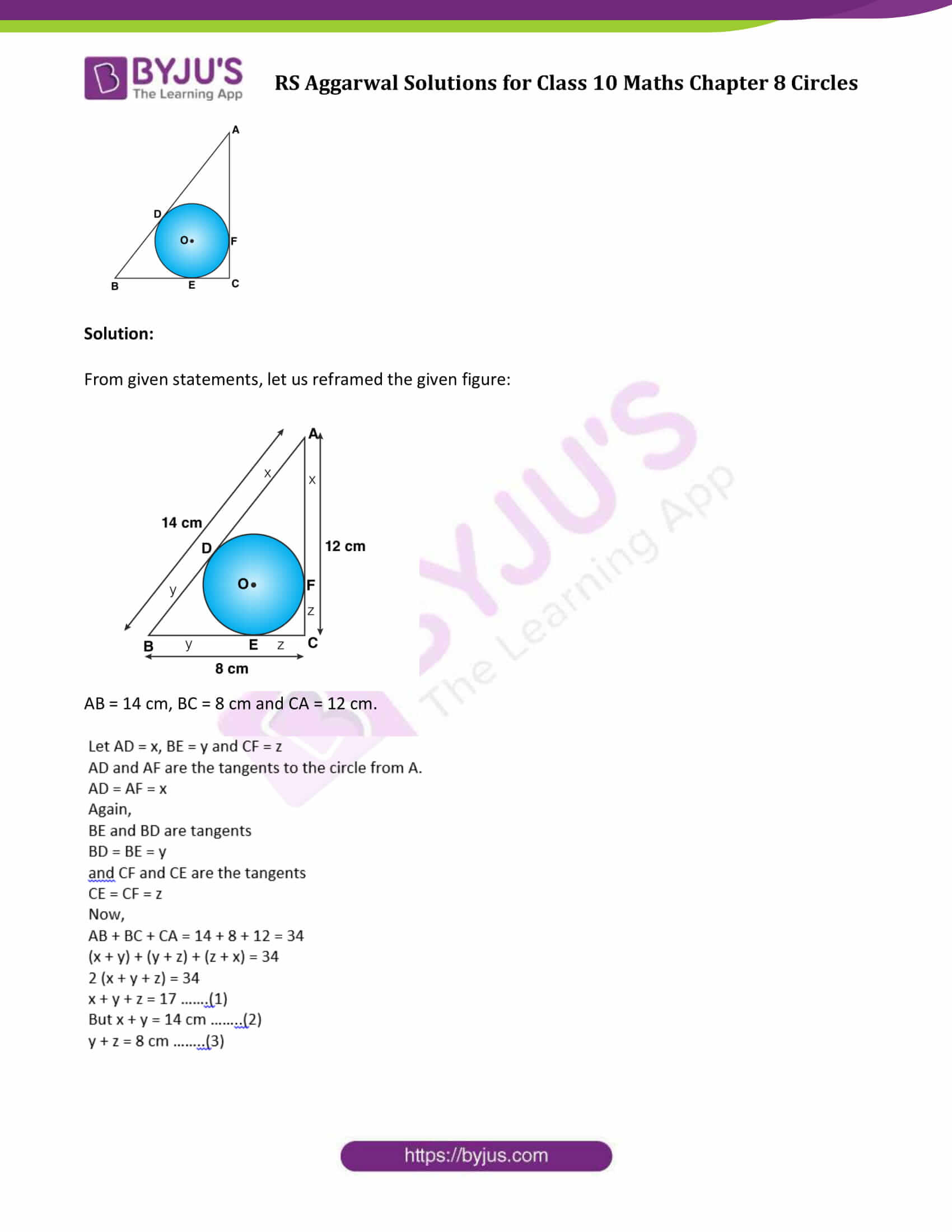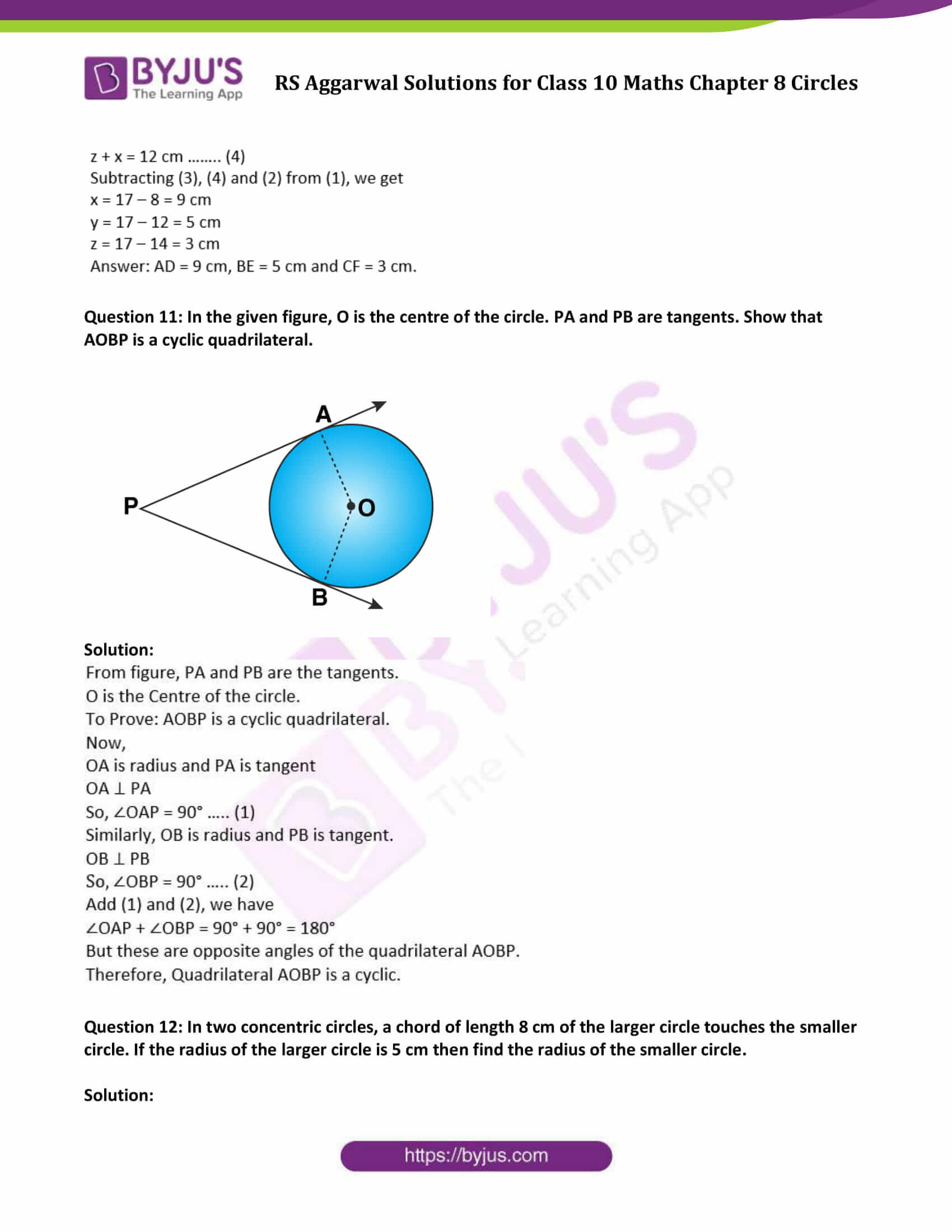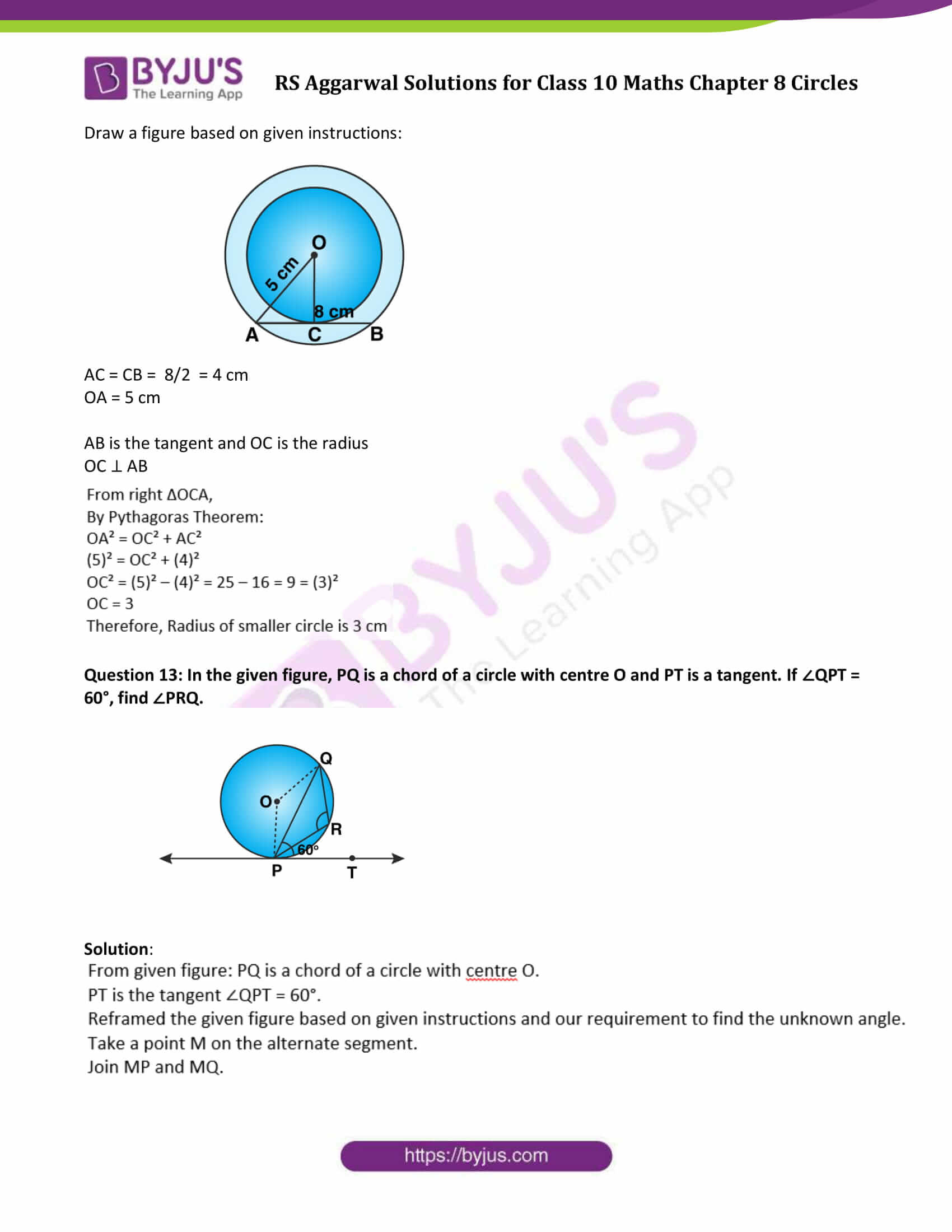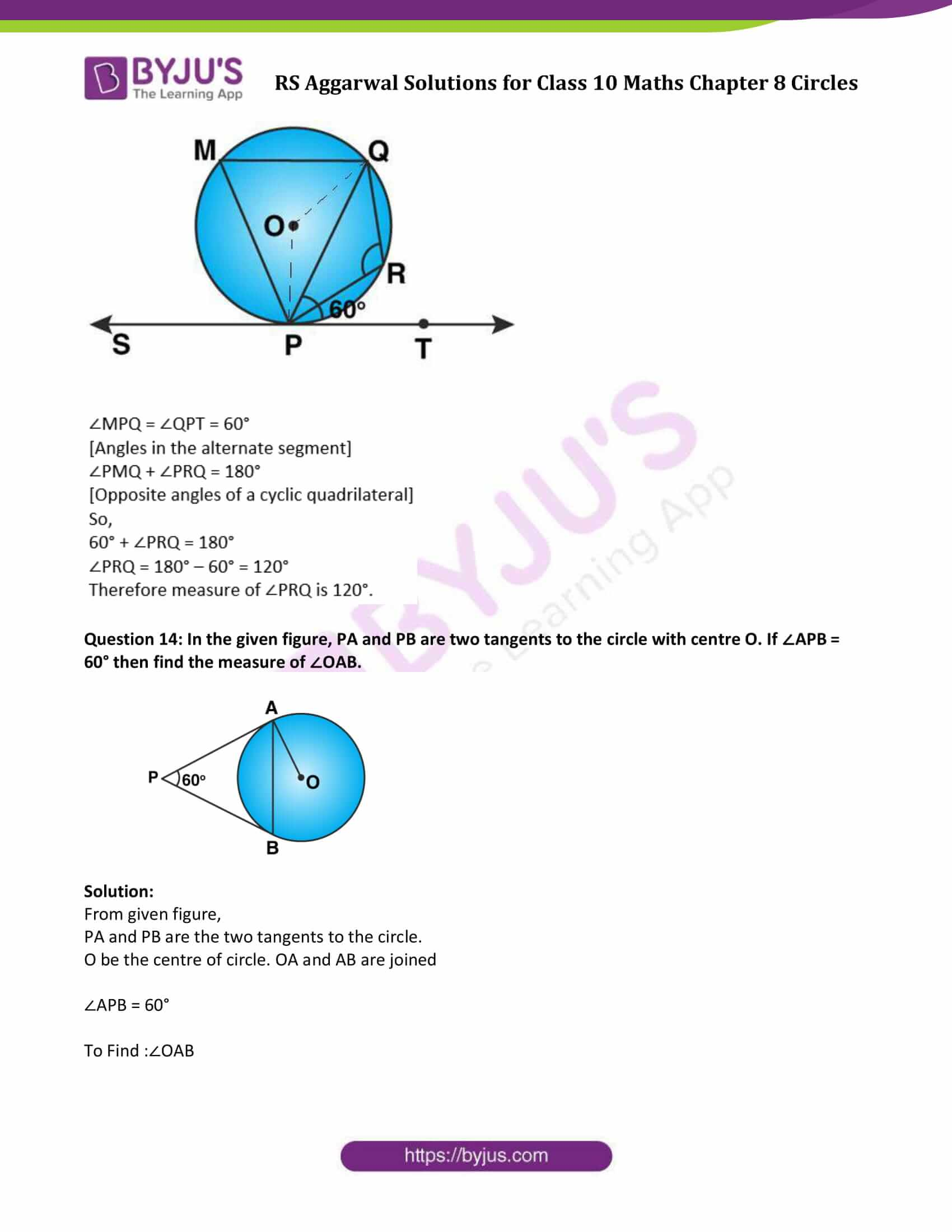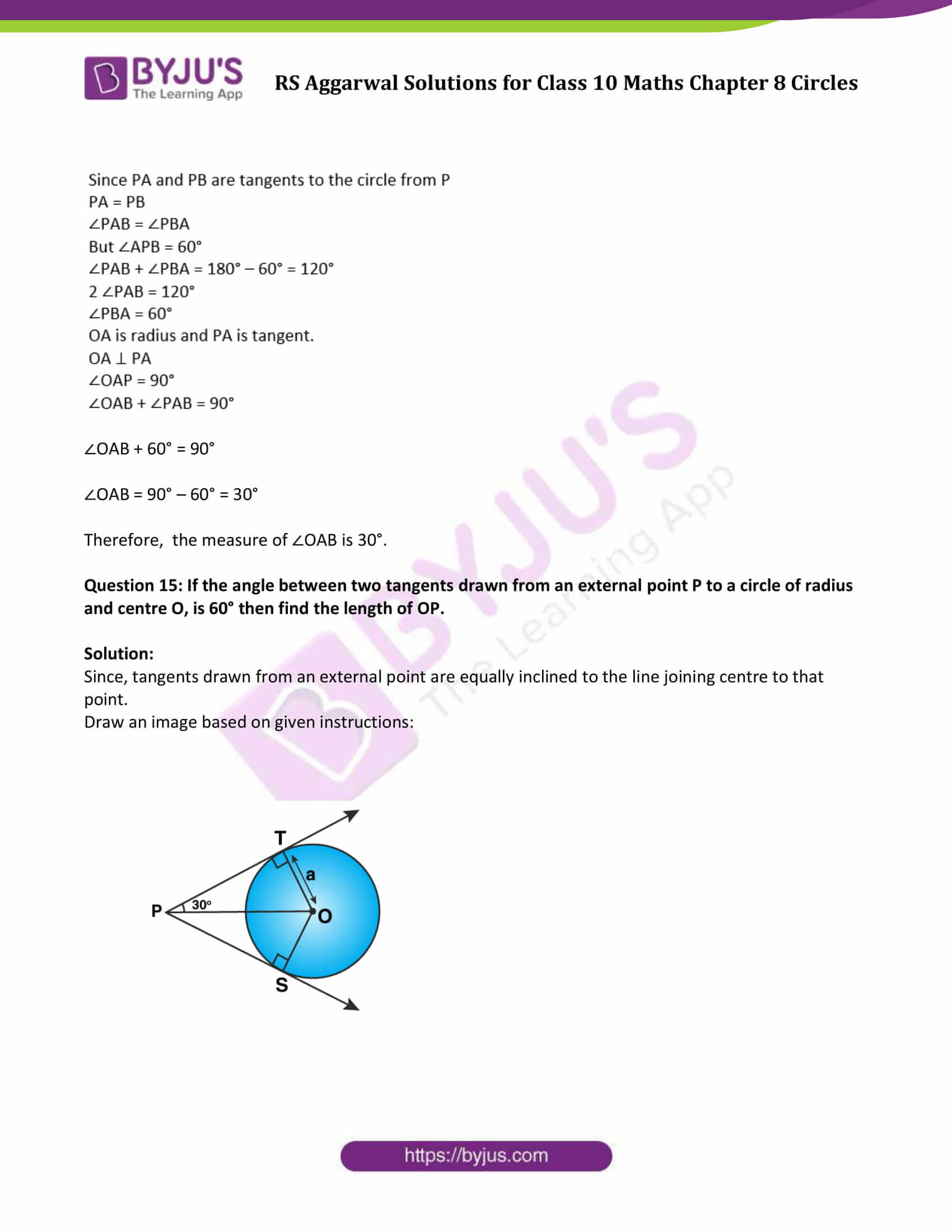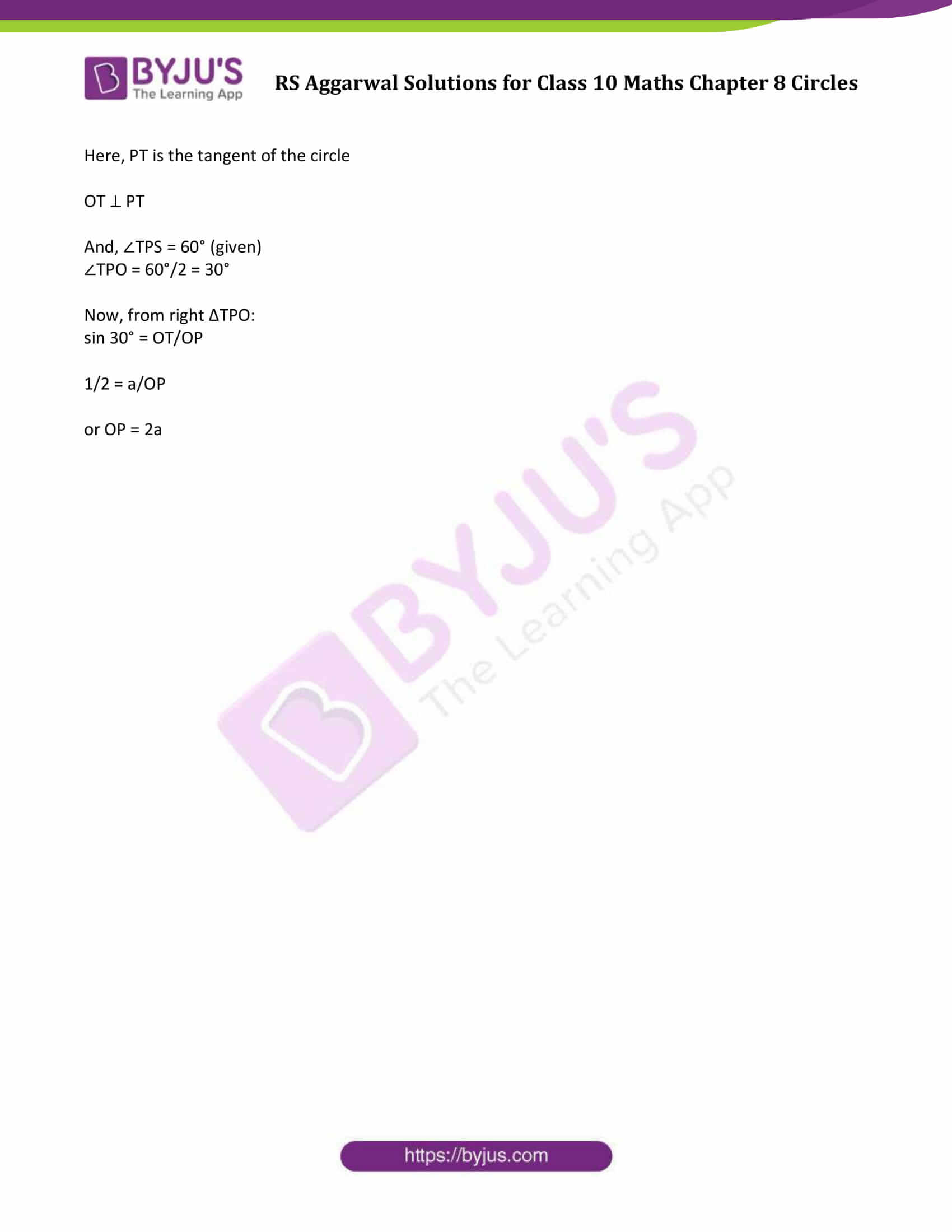### Access Solutions to Maths R S Aggarwal Chapter 8 – Circles

Get detailed solutions for all the questions listed under the below exercises:

Exercise 8A Solutions

Exercise 8B Solutions

## Exercise 8A

Question 1: A point P is at a distance of 29 cm from the centre of a circle of radius 20 cm. Find the length of the tangent drawn from P to the circle.

Solution:

Let O be the center of circle, OP = 29cm (given)

Radius of a circle = 20 cm

Let T be any point on the circumference of the circle, then PT is the tangent to the circle.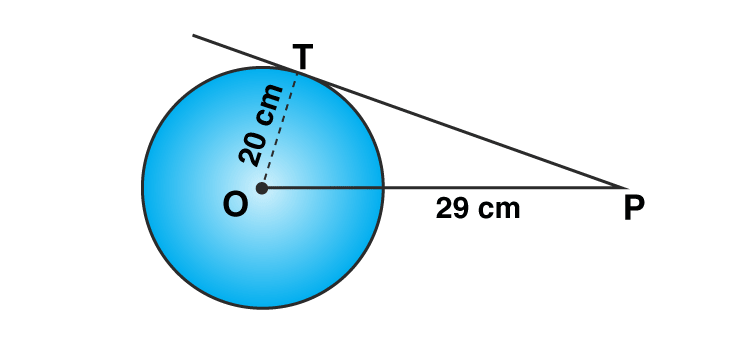From Figure:

OT is radius and PT is the tangent

This implies, OT ⊥ PT

In right ∆OPT,

By Pythagoras Theorem:

OP² = OT² + PT²

(29)² = (20)² + PT²

841 = 400 + PT²

PT² = 441

or PT = 21

Length of tangent PT is 21 cm

Question 2: A point P is 25 cm away from the centre of a circle and the length of tangent drawn from P to the circle is 24 cm. Find the radius of the circle.

Solution:

Let O be the center of circle, OP = 25 cm (given)

Let T be the any point on the circle, then PT = 24 cm

To Find: Radius of the circle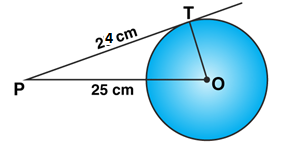From Figure:

OT ⊥ PT

In right ∆OPT,

By Pythagoras Theorem:

OP² = OT² + PT²

25² = OT² + (24)²

625 = OT² + 576

OT = 7

Radius of the circle is 7 cm.

Question 3: Two concentric circles are of radii 6.5 cm and 2.5 cm. Find the length of the chord of the larger circle which touches the smaller circle.

Solution:

Let O be the center of circle. Draw two concentric circles are of radii 6.5 cm and 2.5 cm, and

AB = Chord of the larger circle which touches the smaller circle at C.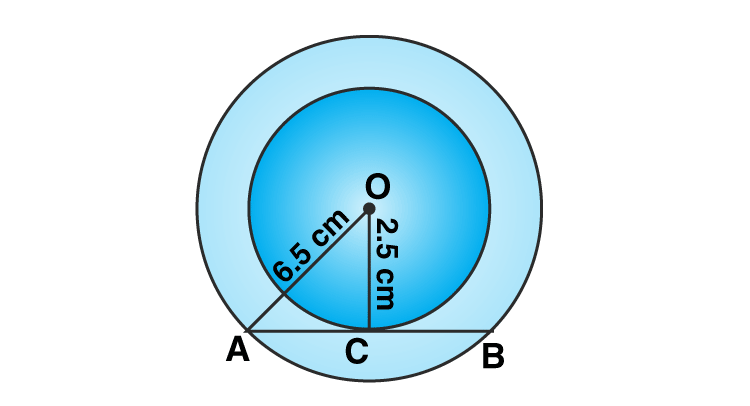From Figure:

OC = radius = 2.5 cm

OA = 6.5 cm

AC = CB

OC ⊥ AB and OC bisects AB at C.

In right ∆OPT,

By Pythagoras Theorem:

OA² = OC² + AC²

6.5² = 2.5² + AC²

42.25 = 6.25 + AC²

AC = 6

Length of chord of a circle = AB = 2xAC = 2 x 6 = 12 cm.

Question 4: In the given figure, a circle inscribed in a triangle ABC, touches the sides AB, BC and AC at points D, E and F respectively. If AB = 12 cm, BC = 8 cm and AC = 10 cm, find the lengths of AD, BE and CF.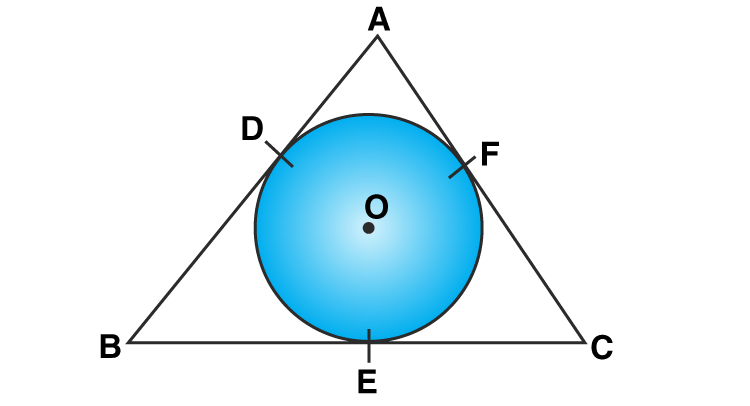Solution: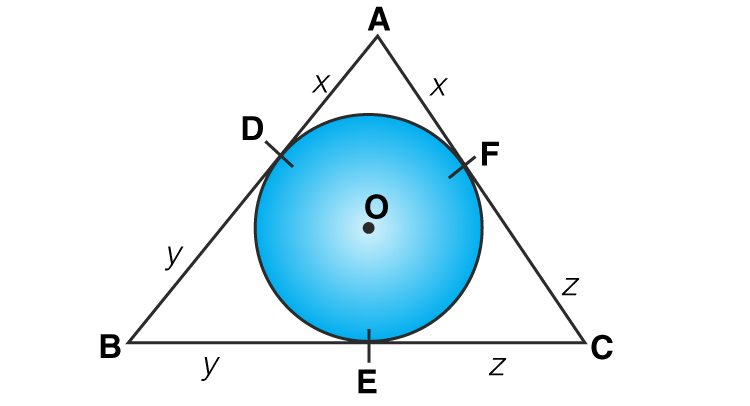AB = 12 cm, BC = 8 cm and AC = 10 cm

To Find: Lengths of AD, BE and CF

AD and AF are tangents to the circle from A.

Similarly, BD and BE are tangents to the circle.

BD = BE = y

and CE and CF are tangents to the circle

CE = CF = z

x + y + 12 ……(1)

y + z = 8 …..(2)

z + x = 10 …..(3)

Adding (1), (2) and (3), we get

2(x + y + z) = 12 + 8 + 10 = 30

x + y + z = 15 …..(4)

Subtract (1) from (4): z = 3

Subtract (2) from (4): x = 7

Subtract (3) from (4): y = 5

Therefore,

AD = 7 cm, BE = 5 cm and CF = 3 cm

Question 5: In the given figure, PA and PB are the tangent segments to a circle with centre O. Show that the points A, O, B and P are concyclic.

Solution:

PA and PB are tangents to a circle with center O (given)

To show: Points A, O, B and P are concyclic.

Since OB ⏊ PB and OA ⏊ AP

∠OBP = ∠OAP = 90°

∠OBP + ∠OAP = 90 + 90 = 180°

[Sum of opposite angles in a quadrilateral is 180°]

AOBP is a cyclic quadrilateral, thus A, O, B and P are concyclic.

Hence proved.

Question 6: In the given figure, the chord AB of the larger of the two concentric circles, with centre O, touches the smaller circle at C. Prove that AC = CB.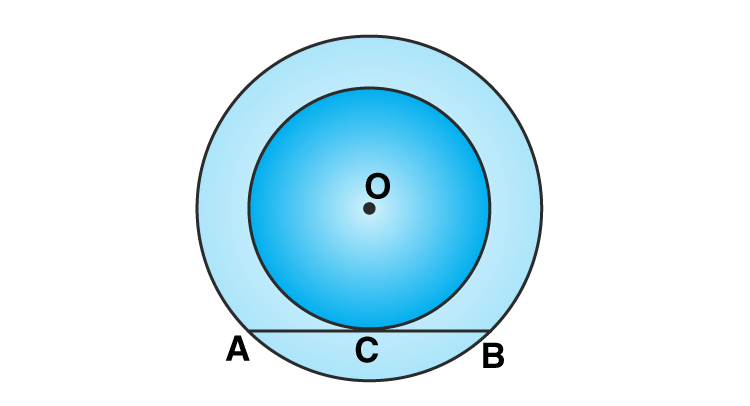Solution:

In the given figure, chord AB of larger circle of the two concentric circles with centre O, touches the smaller circle at C.

To Prove: AC = CB

Join OC, OA and OB.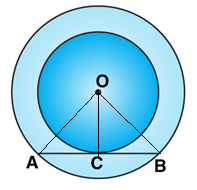Now,

AB is tangent to the smaller circles and OC is the radius.

this implies, OC ⊥ AB.

We have two right angled triangles: ∆OAC and ∆OBC

Here OA = OB = radius of same circle

Side OC = OC = common among both the triangles

∆OAC = ∆OBC (RHS axiom)

By c.p.c.t.

AC = CB

Hence proved.

Question 7: From an external point P, tangents PA and PB are drawn to a circle with, centre O. If CD is the tangent to the circle at a point E and PA = 14 cm, find the perimeter of ∆PCD.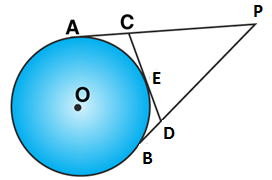Solution:

In the given figure,

CD is a tangent at E and

PA = 14 cm.

Now,

PA and PB are tangents from P to the circle.

PA = PB ….(1)

CA and CE are tangents to the circle.

CA = CE ….(2)

Similarly, BD and DE are tangents to the circle.

DB = DE

Now, perimeter of ∆PCD = Sum of all the sides

= PC + CD + PD

= PC + (CE + ED) + PD

[Using (1) and (2)]

= (PC + CA) + (BD + PD)

= PA + PB

= 14 + 14

= 28

Therefore, perimeter of ∆PCD is 28 cm

Question 8: A circle is inscribed in a ∆ABC touching AB, BC and AC are P, Q and R respectively. If AB = 10 cm, AR = 7 cm and CR = 5 cm, find the length of BC.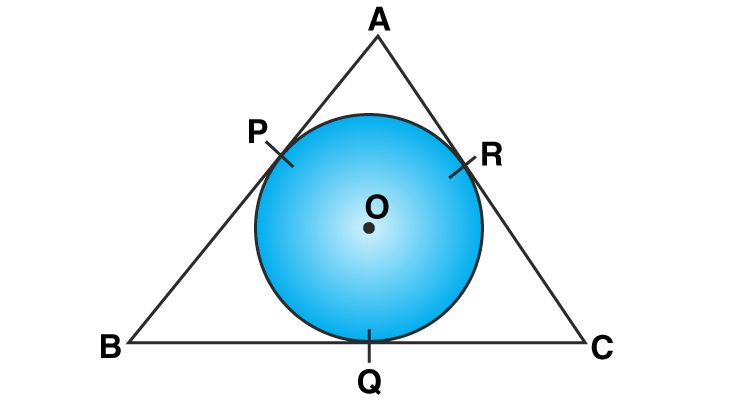Solution:

AB = 10 cm, AR = 7 cm and CR = 5 cm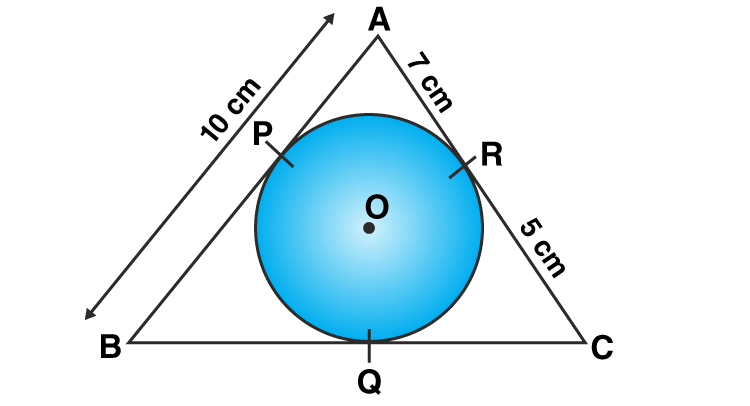AP and AR are the tangents to the circle

AP = AR = 7 cm

From figure: BP = AB – AP = 10 – 7 = 3 cm

Again, BP and BQ are the tangents to the circle, we have

BQ = BP = 3 cm

Similarly, CQ = CR = 5 cm

Now,

BC = CQ + BQ = 5 + 3 = 8

The length of BC is 8 cm.

Question 9: In the given figure, a circle touches all the four sides of a quadrilateral ABCD whose three sides are AB = 6 cm, BC = 7 cm and CD = 4 cm. Find AD.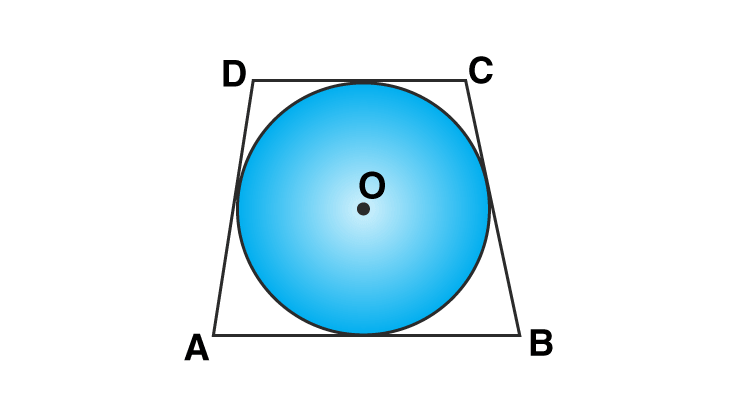Solution:

Here: AB = 6 cm, BC = 7 cm and CD = 4 cm

Let P, Q, R and S are 4 points touches the sides of a quadrilateral.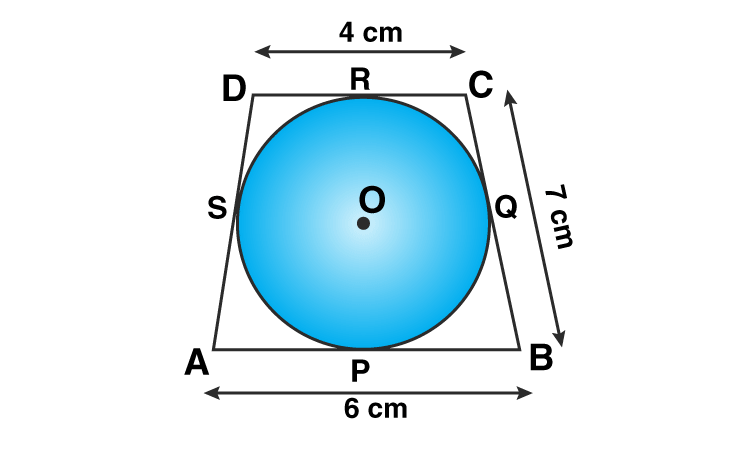We know, tangents from an external point to a circle are always equal.

Therefore, AP = AS, BP = BQ, CR = CQ and DR = DS

Now from figure, AP + BP + CR + DR = AS + BQ + CQ + DC

(AP + BP) + (CR + DR) = (AS + DS) + (BQ + CQ)

AB + CD = AD + BC

6 + 4 = AD + 7

Question 10: In the given figure, an isosceles triangle ABC, with AB = AC, circumscribes a circle. Prove that the point of contact P bisects the base BC.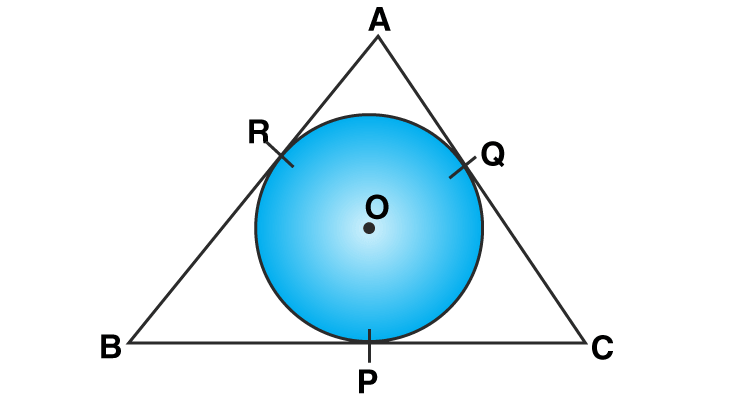Solution:

∆ABC is an isosceles triangle where AB = AC and circumscribed a circle.

The circle touches its sides BC, CA and AB at P, Q and R respectively.

To Prove : P bisects the base BC, i.e. BP = PC

Now form figure,

BR and BP are tangents to the circle.

So, BR = BP …..(1)

AR and AQ are tangents to the circle.

AR = AQ But AB = AC

AB – AR = AC – AQ

BR = CQ …..(2)

Similarly, CP and CQ are tangents to the circle.

CP = CQ ……(3)

From (1), (2) and (3)

BP = PC

Hence Proved.

Question 11: In the given figure, O is the centre of two concentric circles of radii 4 cm and 6 cm respectively. PA and PB are tangents to the outer and inner circle respectively. If PA = 10 cm, find the length of PB up to one place of decimal.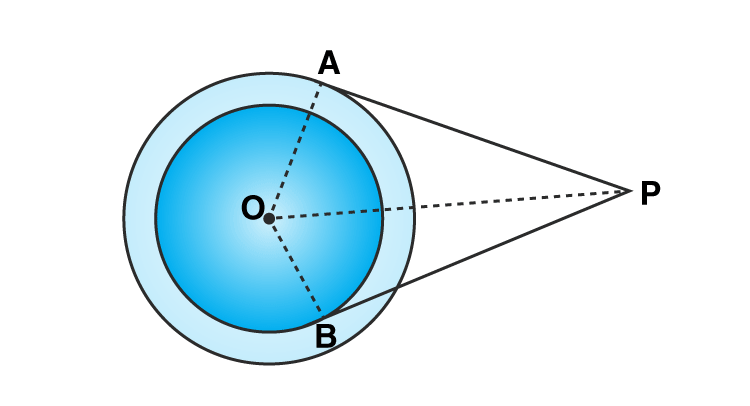Solution:

In the given figure,

PA and PB are the tangents drawn from P, to the outer circle and inner circle respectively.

PA = 10 cm

OA and OB are the radii and

PA and PB are two tangents to the circles respectively

So,

OA ⊥ PA and OB ⊥ PB

In right ∆OAP,

By Pythagoras Theorem:

OP² = OA² + PA²

= (6)² + (10)²

OP²= 136 …(1)

From right ∆OBP,

OP² = OB² + PB²

136 = (4)² + PB²

136 = 16 + PB²

[Using equation (1)]

We have PB² = 136 – 16 = 120

Or PB = √120 cm = 2√30 cm = 2 x 5.47 = 10.9

Answer: Length of PB is 10.9 cm

Question 12: In the given figure, a triangle ABC is drawn to circumscribe a circle of radius 3 cm such that the segments BD and DC into which BC is divided by the point of contact D, are of lengths 6 cm and 9 cm respectively. If the area of ∆ABC = 54 cm² then find the lengths of sides AB and AC.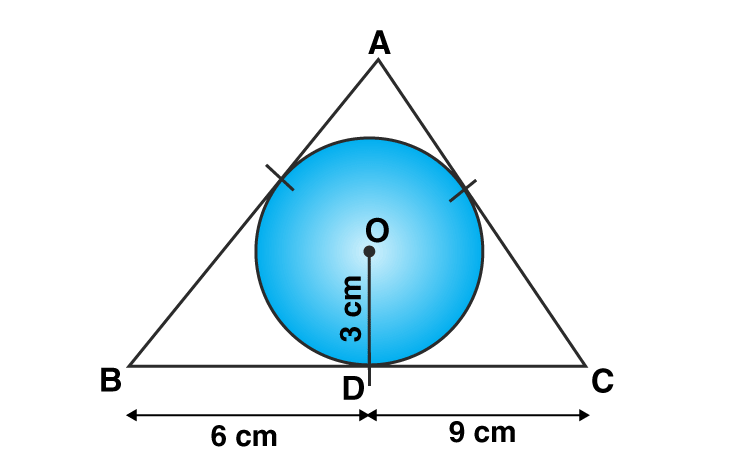Solution: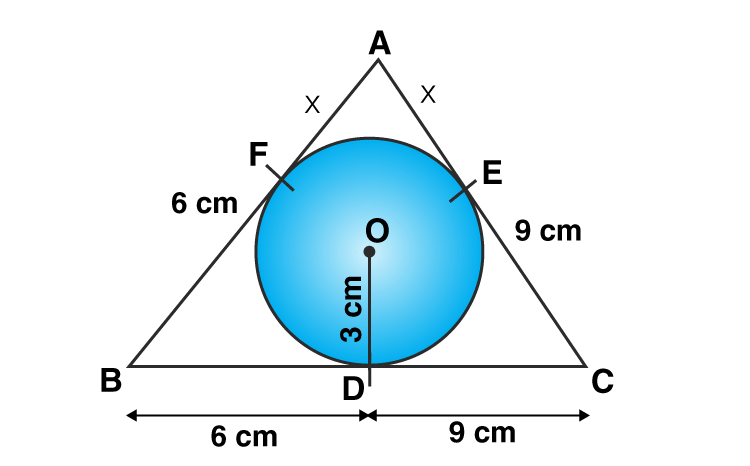In the given figure, ∆ABC circumscribed the circle with centre O.

BD = 6 cm, DC = 9 cm

Area of ∆ABC = 54 cm²

To find : Lengths of AB and AC.

AF and EA are tangents to the circle at point A.

Let AF = EA = x

BD and BF are tangents to the circle at point B.

BD = BF = 6 cm

CD and CE are tangents to the circle at point C.

CD = CE = 9 cm

Now, new sides of the triangle are:

AB = AF + FB = x + 6 cm

AC = AE + EC = x + 9 cm

BC = BD + DC = 6 + 9 = 15 cm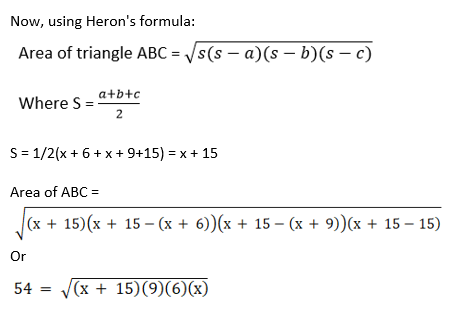Squaring both sides, we have

54^2 = 54x(x + 15)

x^2 + 15x – 54 = 0

Solve this quadratic equation and find the value of x.

x^2 + 18x – 3x – 54 = 0

x(x + 18) – 3(x + 18) = 0

(x – 3)(x + 18) = 0

Either x = 3 or x = – 18

But x cannot be negative.

So, x = 3

AB = x + 6 = 3 + 6 = 9 cm

AC = x + 9 = 3 + 9 = 12 cm

Question 13: PQ is a chord of length 4.8 cm of a circle of radius 3 cm. The tangents at P and Q intersect at a point T as shown in the figure. Find the length of TP.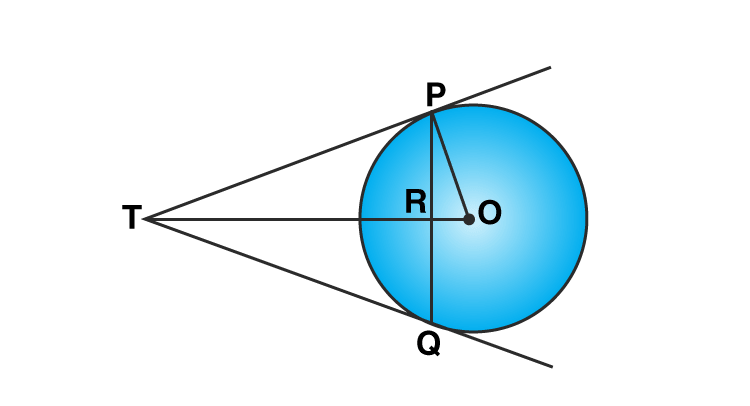Solution:

Radius of the circle is 3 cm and PQ = 4.8 cm.

PQ is a chord of the circle with centre O.

The tangents at P and Q intersect at point T

So, TP and TQ are tangents

OP and OT are joined.

Join OQ

We have two right triangles: △OPT and △OQT

Here,

OT = OT (Common)

PT = QT (tangents of the circle)

OP = OQ (radius of the same circle)

By Side – Side – Side Criterion

△OPT ≅△OQT

Therefore, ∠POT = ∠OQT

Again, from triangles △OPR and △OQR

OR = OR (Common)

OP = OQ (radius of the same circle)

∠POR = ∠OQR (from above result)

By Side – Angle – Side Criterion

△OPR ≅△OQT

Therefore, ∠ORP = ∠ORQ

Now,

∠ORP + ∠ORQ = 180°

(Sum of linear angles = 180 degrees)

∠ORP + ∠ORP = 180°

∠ORP = 90°

This implies, OR ⏊ PQ and RT ⏊ PQ

Also OR perpendicular from center to a chord bisects the chord,

PR = QR = PQ/2 = 4.8/2 = 2.4 cm

Applying Pythagoras Theorem on right triangle △OPR,

(OP)^2 = (OR)^2 + (PR)^2

(3)^2 = (OR)^2 + (2.4)^2

OR = 1.8 cm

Applying Pythagoras Theorem on right angled △TPR,

(PT)^2 = (PR)^2 + (TR)^2 …(1)

Also, OP ⏊ OT

Applying Pythagoras Theorem on right △OPT,

(PT)^2 + (OP)^2 = (OT)^2

[(PR)^2 + (TR)^2 ] + (OP)^2 = (TR + OR)^2

(Using equation (1) and from figure)

(2.4)^2 + (TR)^2 + (3)^2 = (TR + 1.8)^2

4.76 + (TR)^2 + 9 = (TR)^2 + 2(1.8)TR + (1.8)^2

13.76 = 3.6 TR + 3.24

TR = 2.9 cm [approx.]

From (1) => PT^2 = (2.4)^2 + (2.9)^2

PT^2 = 4.76 + 8.41

or PT = 3.63 cm [approx.]

Answer: Length of PT is 3.63 cm.

Question 14: Prove that the line segment joining the points of contact of two parallel tangents of a circle, passes through its centre.

Solution:

Let O is the centre of the circle.

Let PQ and RS are two parallel tangents which touches the circle at points A and B.

OA and OB are joined.

To Prove : AB passes through point O.

Draw OC || RS ||PQ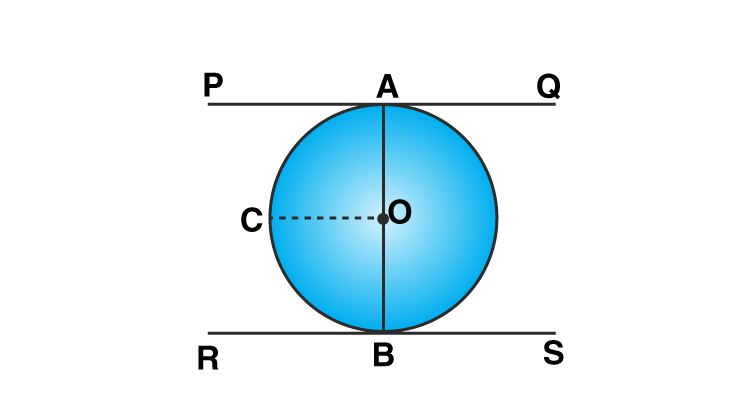Now,

OA = OB = Radius and

PQ is tangent of circle passing through A

OA ⊥ PQ

Which implies, ∠OAP = 90°

RS is the tangent of circle passing through B

OB ⊥ RS

Which implies, ∠OBR = 90°

Since PQ || OC

∠AOC + ∠OAP = 180° (Co-interior angles)

∠AOC + 90° = 180°

∠AOC = 180° – 90° = 90°

Similarly, ∠BOC = 90°

∠AOC + ∠BOC = 90° + 90° = 180°

AOB is a straight line. Therefore, AB passes through the centre of the circle.

Question 15: In the given figure, a circle with centre O, is inscribed in a quadrilateral ABCD such that it touches the side BC, AB, AD and CD at points P, Q, R and S respectively. If AB = 29 cm, AD = 23 cm, ∠B = 90° and DS = 5 cm then find the radius of the circle.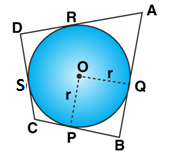Solution:

In the given figure,

O be the centre of circle which is inscribed in a quadrilateral ABCD.

And OP = OQ = r = radius of circle

The circle touches the sides of quadrilateral at P, Q, R and S respectively.

AB = 29 cm, AD = 23 cm, ∠B = 90°

DS = 5 cm

Join OP and OQ.

Now,

OP = OQ = r and ∠B = 90°

So, PBQO is a square.

DR and DS are the tangents to the circle.

DR = DS = 5 cm

AQ and AR are tangents to the circle.

AR = AD – DR = 23 – 5 = 18 cm

AQ = AR = 18 cm

And BQ = AB – AQ = 29 – 18 = 11 cm

Since PBQO is a square.

OP = OQ = BQ = 11 cm

Hence, radius of the circle is 11 cm

Question 16: In the given figure, O is the centre of the circle and TP is the tangent to the circle from an external point T. If ∠PBT = 30°, prove that BA : AT = 2 : 1.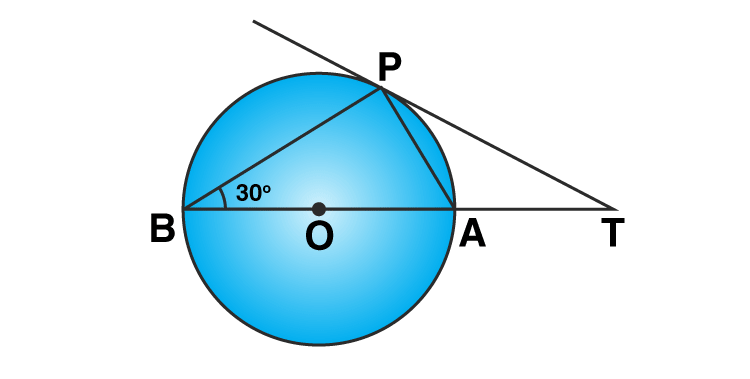Solution:

In the given figure,

TP is the tangent from an external point T and

∠PBT = 30°

Now,

∠APB = 90° (Angle in a semicircle)

∠PBT = 30° (given)

So, ∠PAB = 90° – 30° = 60°

But, ∠PAT + ∠PAB = 180° (Linear pair)

∠PAT + 60° = 180°

∠PAT = 180° – 60° = 120°

Also, ∠APT = ∠PBA = 30° (Angles in the alternate segment)

In ∆PAT,

∠PTA = 180° – (120° + 30°) = 180° – 150° = 30°

PA = AT

In right ∆APB,

sin 30^0 = AP/AB

1/2 = AP/AB

AB = 2 AP

Since AP = AT

AB = 2 AT

or AB/AT = 2/1

AB:AT = 2:1

or BA:AT = 2:1. Hence Proved.

## Exercise 8B

Question 1: In the adjoining figure, a circle touches all the four sides of a quadrilateral ABCD whose sides are AB = 6 cm, BC = 9 cm and CD = 8 cm. Find the length of side AD.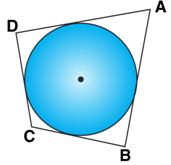Solution:

In the given figure,

A circle touches all the four sides of a quadrilateral ABCD whose sides are AB = 6 cm, BC = 9 cm and CD = 8 cm

Let P, Q, R and S are points where circle touches the sides AB, BC, CD and DA respectively.

Therefore,

AB + CD = BC + AD

6 + 8 = 9 + AD

AD = 14 – 9 = 5

Length of AD is 5 cm.

Question 2: In the given figure, PA and PB are two tangents to the circle with centre O. If ∠APB = 50° then what is the measure of ∠OAB.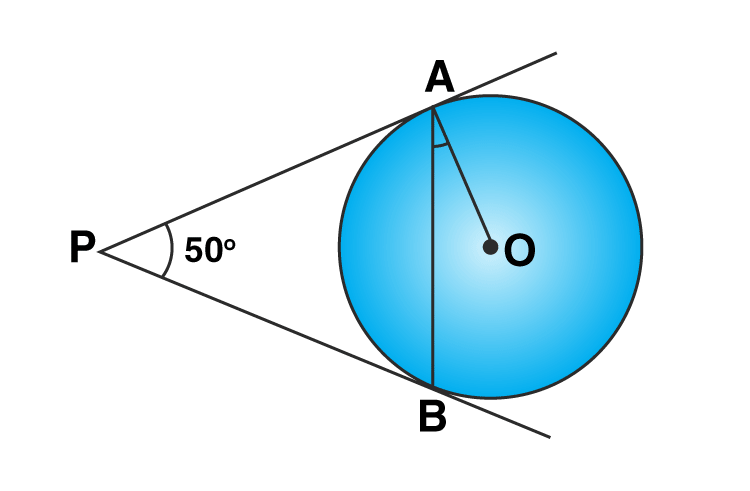Solution:

In the given figure,

∠APB = 50°

PA and PB are two tangents to the circle with centre O.

Join OB.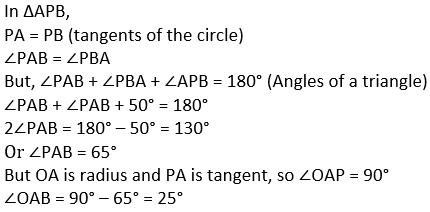Question 3: In the given figure, O is the centre of a circle. PT and PQ are tangents to the circle from an external point P. If ∠TPQ = 70°, find ∠TRQ.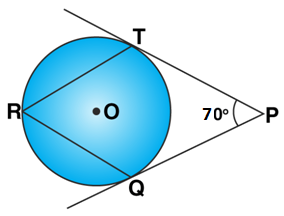Solution:

Here, O is the centre of circle.

PQ and PT are tangents to the circle from a point P

R is any point on the circle. RT and RQ are joined.

∠TPQ = 70°

Now,

Join TO and QO.

∠TOQ = 180° – 70° = 110°

Here, OQ and OT are perpendicular on QP and TP.

∠TOQ is on the centre and ∠TRQ is on the rest part.

∠TRQ = 1/2∠TOQ = ½( 110°) = 55°

Therefore, ∠TRQ = 55 degrees.

Question 4: In the given figure, common tangents AB and CD to the two circles with centres O1 and O2 intersect at E. Prove that AB = CD.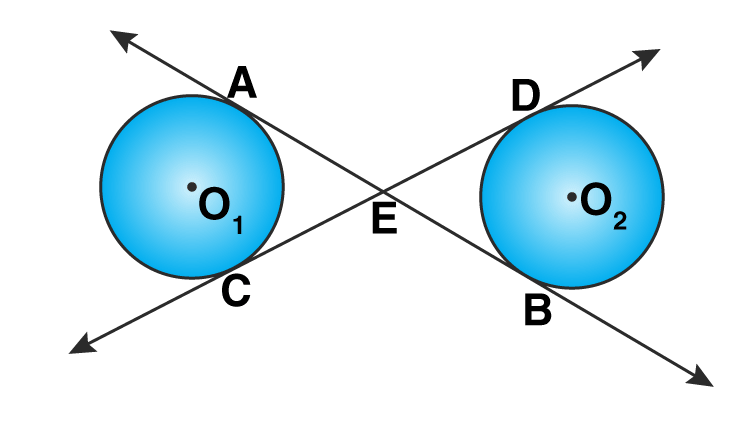Solution:

In the given figure, common tangents

AB and CD are common tangents to the two circles with centres O1 and O2 intersecting at point E.

Circle O1:

EA and EC are tangents

EA = EC …(1)

Circle O2:

EB and ED are tangents

EB = ED …(2)

EA + EB = EC + ED

AB = CD

Hence proved.

Question 5: If PT is a tangent to a circle with centre O and PQ is a chord of the circle such that ∠QPT = 70°, then find the measure of ∠POQ.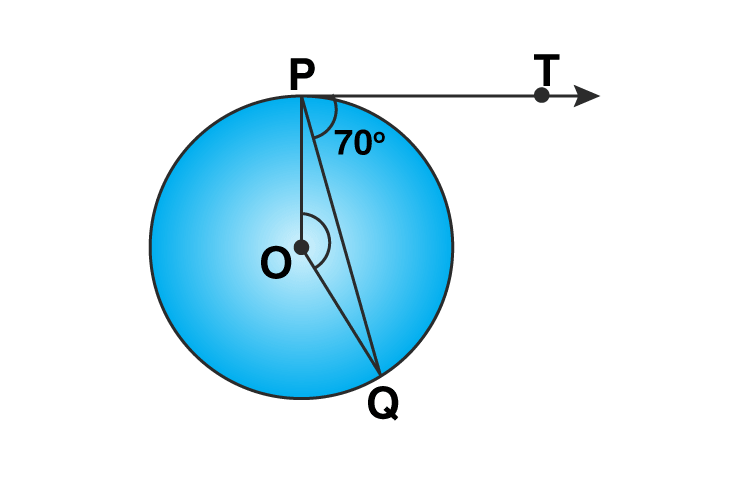Solution: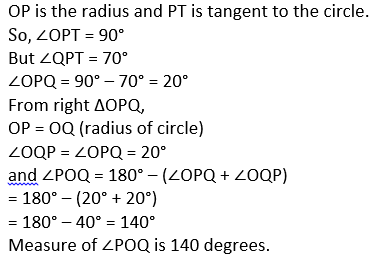Question 6: In the given figure, a triangle ABC is drawn to circumscribe a circle of radius 2 cm such that the segments BD and DC into which BC is divided by the point of contact D, are of lengths 4 cm and 3 cm respectively. If the area of ∆ABC = 21 cm² then find the lengths of sides AB and AC.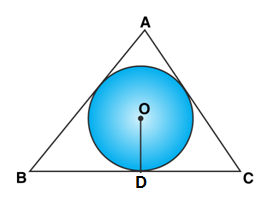Solution:

Given:

∆ABC is circumscribed a circle with centre O and radius 2 cm.

Point D divides BC as

BD = 4 cm, DC = 3 cm, OD = 2 cm

Area of ∆ABC = 21 cm²

Join OA, OB, OC, OE and OF.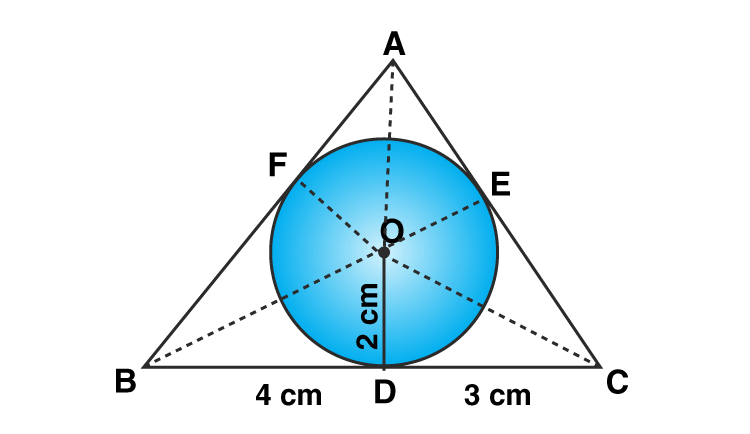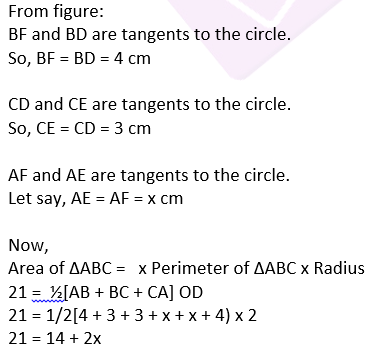x = 3.5

Therefore,

AB = AF + FB = 3.5 + 4 = 7.5 cm

AC = AE + CE = 3.5 + 3 = 6.5 cm

Question 7: Two concentric circles are of radii 5 cm and 3 cm. Find the length of the chord of the larger circle (in cm) which touches the smaller circle.

Solution:

Two concentric circles are of radii 5 cm and 3 cm.

Let O be the centre of circle.

Let AB is chord of larger circle which touches the smaller circle at C.

Join OA and OC.

Below is the figure: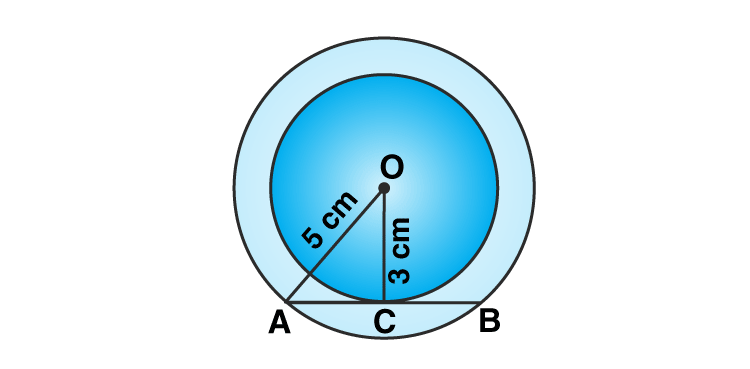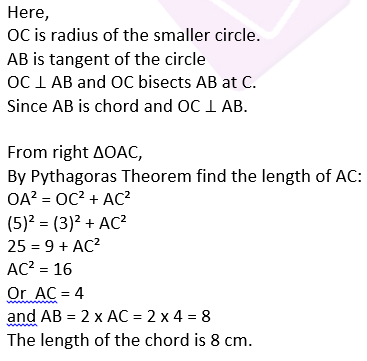Question 8: Prove that the perpendicular at the point of contact of the tangent to a circle passes through the centre.

Solution:

AB is the tangent to the circle at point P

Let O be the centre.

PL ⊥ AB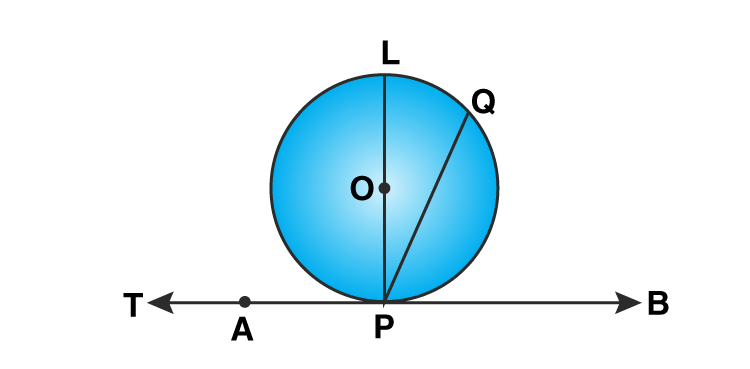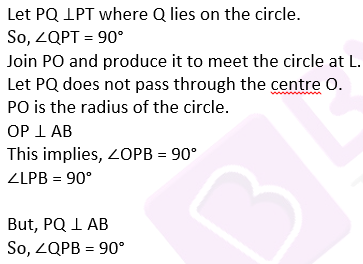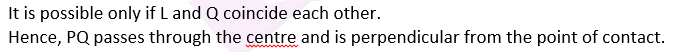Question 9: In the given figure, two tangents RQ and RP are drawn from an external point R to the circle with centre O. If ∠PRQ = 120°, then prove that OR = PR + RQ.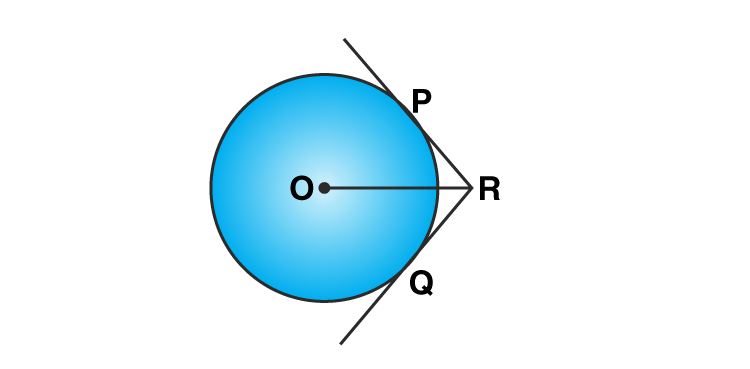Solution: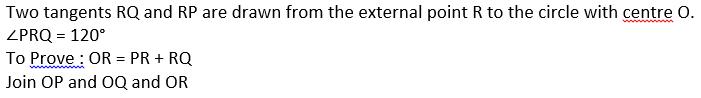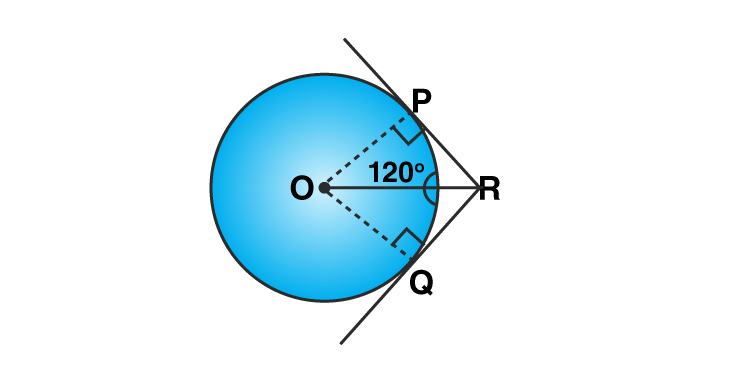∠PRQ = ∠QRO = 120°/2 = 60°

RQ and RP are the tangent to the circle.

OQ ⊥ QR and OP ⊥ PR

Form right ∆OPR,

∠POR = 180° – (90° + 60°) = 30°

and∠QOR = 30°

cos a = PR/OR (suppose ‘a’ be the angle)

cos 60° =PR/OR

1/2 = PR/OR

OR = 2 PR

Again from right ∆OQR,

OR = 2 QR

From both the results, we have

2 PR + 2 QR = 2OR

or OR = PR + RQ

Hence Proved.

Question 10: In the given figure, a circle inscribed in a triangle ABC touches the sides AB, BC and CA at points D, E and F respectively. If AB = 14 cm, BC = 8 cm and CA = 12 cm. Find the lengths AD, BE and CF.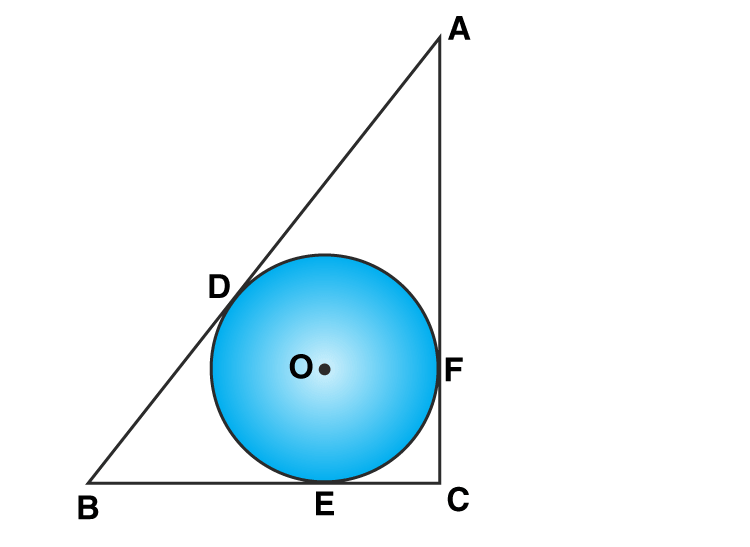Solution:

From given statements, let us reframed the given figure: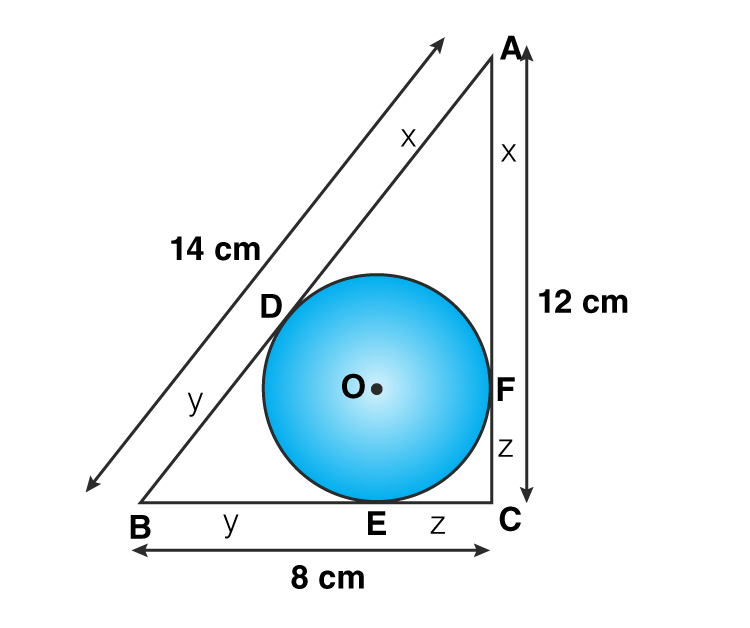AB = 14 cm, BC = 8 cm and CA = 12 cm.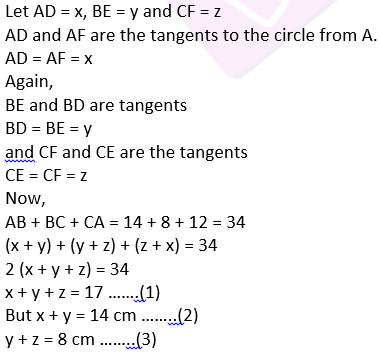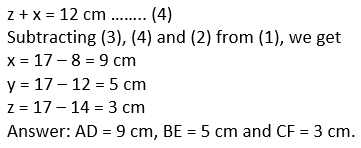Question 11: In the given figure, O is the centre of the circle. PA and PB are tangents. Show that AOBP is a cyclic quadrilateral.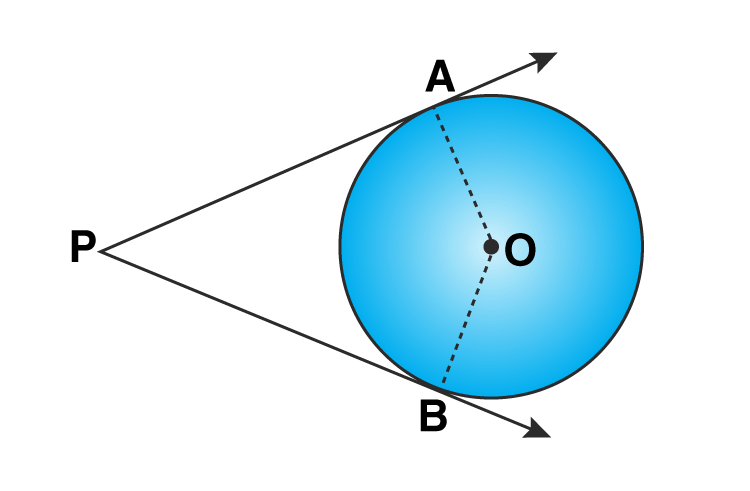Solution: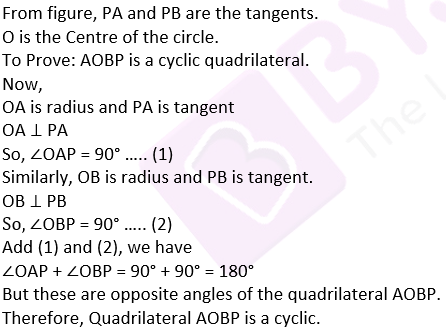Question 12: In two concentric circles, a chord of length 8 cm of the larger circle touches the smaller circle. If the radius of the larger circle is 5 cm then find the radius of the smaller circle.

Solution:

Draw a figure based on given instructions: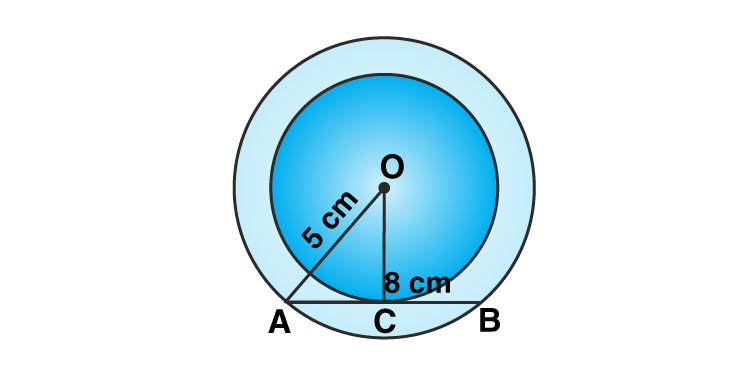AC = CB = 8/2 = 4 cm

OA = 5 cm

AB is the tangent and OC is the radius

OC ⊥ AB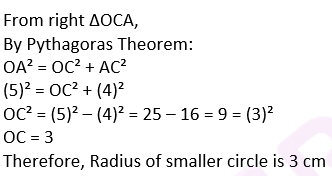Question 13: In the given figure, PQ is a chord of a circle with centre O and PT is a tangent. If ∠QPT = 60°, find ∠PRQ.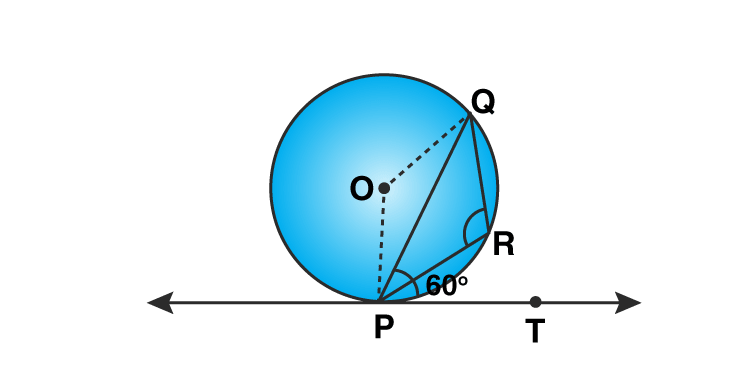Solution: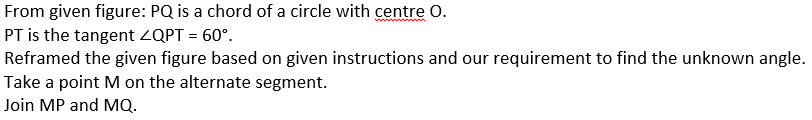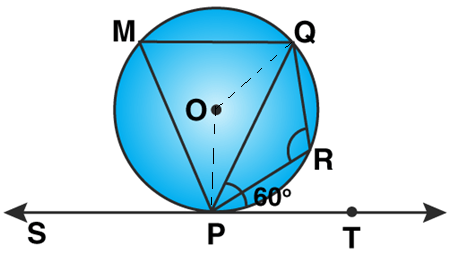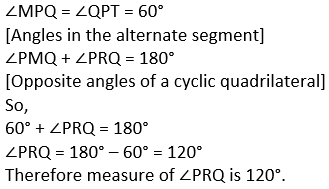Question 14: In the given figure, PA and PB are two tangents to the circle with centre O. If ∠APB = 60° then find the measure of ∠OAB.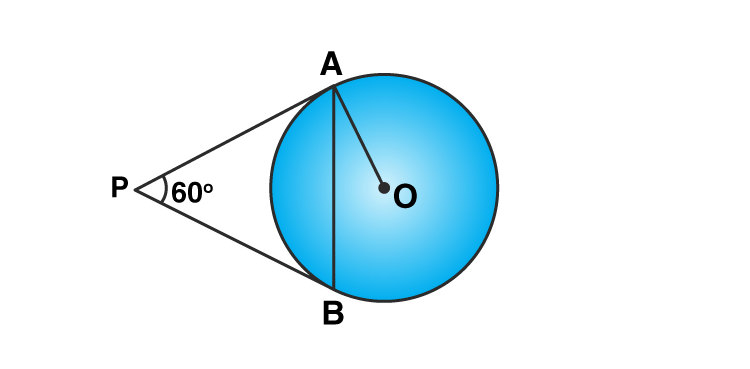Solution:

From given figure,

PA and PB are the two tangents to the circle.

O be the centre of circle. OA and AB are joined

∠APB = 60°

To Find :∠OAB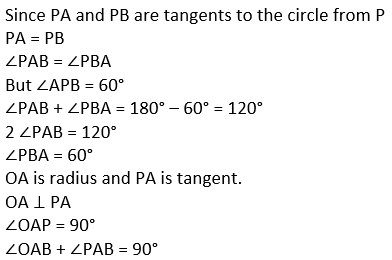∠OAB + 60° = 90°

∠OAB = 90° – 60° = 30°

Therefore, the measure of ∠OAB is 30°.

Question 15: If the angle between two tangents drawn from an external point P to a circle of radius and centre O, is 60° then find the length of OP.

Solution:

Since, tangents drawn from an external point are equally inclined to the line joining centre to that point.

Draw an image based on given instructions: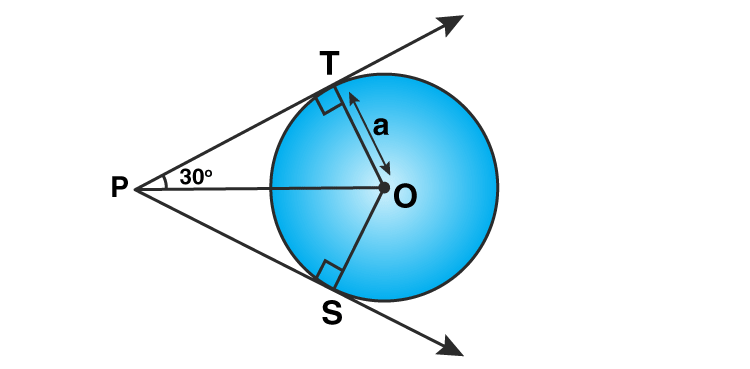Here, PT is the tangent of the circle

OT ⊥ PT

And, ∠TPS = 60° (given)

∠TPO = 60°/2 = 30°

Now, from right ∆TPO:

sin 30° = OT/OP

1/2 = a/OP

or OP = 2a

## R S Aggarwal Solutions for Chapter 8 Circles

In this chapter students will study important concepts on Circles as listed below:

• Circles introduction
• Secant
• Tangent, length of a tangent and number of tangents to a circle.
• Important theorems on circle tangents.

### Key Features of R S Aggarwal Solutions for Class 10 Maths Chapter 8 Circles

1. R S Aggarwal Solutions covers all the exercise questions.

2. All questions are solved by subject experts.

3. Easy for quick revision.

4. These solutions will be useful for Olympiads, board exams, and other competitive exams.